ZeitschriftendatenFormat
Zeitschrift
Erstveröffentlichung
09 Nov 2012
Erscheinungsweise
4 Hefte pro Jahr
Sprachen
EnglischOpen Access

# Characteristic parameters of soil failure criteria for plane strain conditions – experimental and semi-theoretical study

###### Akzeptiert: 29 Apr 2021
ZeitschriftendatenFormat
Zeitschrift
Erstveröffentlichung
09 Nov 2012
Erscheinungsweise
4 Hefte pro Jahr
Sprachen
Englisch
Introduction

Many different failure criteria, being part of soil constitutive models, can be found in the literature. The basic one to which all others are usually compared is the Mohr–Coulomb condition, due to its simplicity. It proved its usefulness in classic triaxial compression, where a cylindrical soil sample is subjected to an axisymmetric state of stress (σ1, σ2 = σ3, Fig. 1a). However, the problem of soil strength is more complex when true triaxial stress conditions are considered, and the principal stresses σ1, σ2, σ3 have different values (Fig. 1b).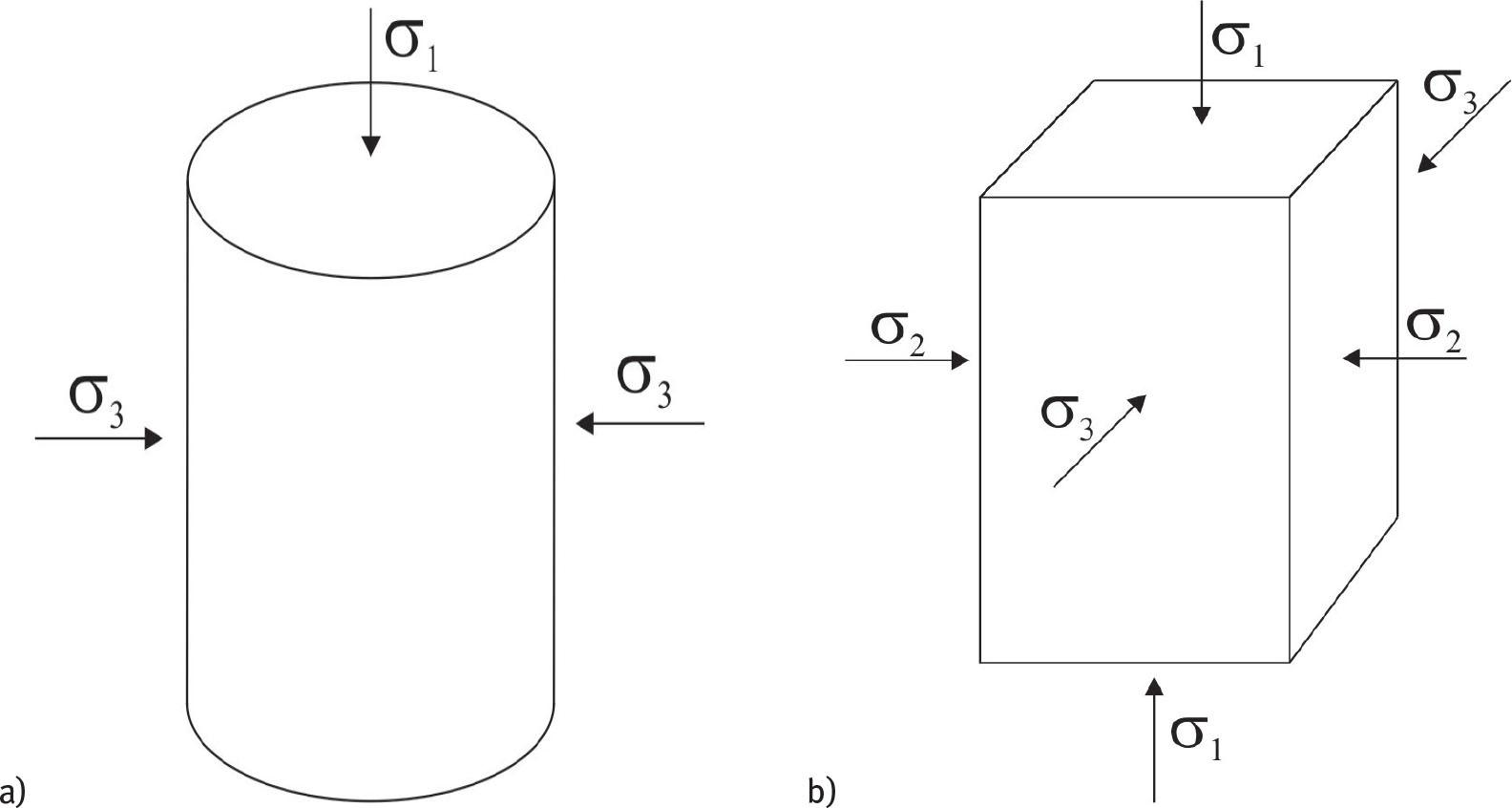Two-dimensional and three-dimensional soil stress states: a) cylindrical sample in axisymmetric stress conditions, σ2 = σ3 and b) rectangular sample in true triaxial conditions, σ1 ≠ σ2 ≠ σ3.

This paper considers the most frequently used isotropic soil failure criteria (yield surfaces): Mohr–Coulomb, Drucker–Prager, Matsuoka–Nakai and Lade–Duncan. The detailed descriptions of the selected criteria are in Drucker and Prager (1952), Lade and Duncan (1975), Matsuoka and Nakai (1974) and Matsuoka and Nakai (1985).

The research on soil failure continues, and except listed above, there are also other criteria proposed in the literature. Georgiadis et al. (2004), Houlsby (1986) or Liu et al. (2012) suggest a yield surface which is a combination of the criteria mentioned above. Lagioia and Panteghini (2014) present a reformulation of the original Matsuoka–Nakai criterion to overcome the limitations which make its use in a stress point algorithm problematic. A novel soil strength criterion, where the cube root of principal stresses is constant, is proposed by Shao et al. (2017), and it shows that the Lade–Duncan criterion is not just an empirical one, as previously thought, but has a physical background.

Mohr–Coulomb failure condition is built on simplifying assumption that soil behaviour is governed by the difference between maximum and minimum principal stress (σ1 - σ3) and does not depend on the intermediate principal stress (σ2). It is clear that such simplification may be valid in some special conditions only. The influence of the intermediate principal stress σ2 on soil shear strength is discussed by Bishop (1971), Kulhawy and Mayne (1990) or Ochiai and Lade (1983). Barreto and O’Sullivan (2012) examined the effect of inter-particle friction (μ) and the intermediate stress σ2 on the granular material's response, using the discrete element method (DEM). They have shown that both parameters significantly affect the strength characteristics. DEM is also used by Li et al. (2017) to describe the behaviour of soil under complex stress state. They observed that the Bishop's parameter b (Eq. (9)) significantly affects the non-coaxial behaviour of granular materials.

The contribution of the intermediate principal stress σ2 to the plane strain soil strength is particularly studied for practical reasons: to analyse longitudinal foundations, slopes, retaining walls and long excavations. Besides, many experimental techniques, like full-field displacement measurements by digital image correlation (DIC), are usually performed on rectangular plane strain models. Also ground flow problems, static liquefaction and instability are studied in plane strain conditions (Wanatowski and Chu, 2007; Wanatowski et al. 2010).

Experimental investigations of dense granular soils have shown that the plane strain shear strength is higher than that in the axisymmetric conditions (Alshibli et al., 2003). In the case of loose soils, there is no such difference; see Cornforth (1964), Lee (1970), Rowe (1969) and Schanz and Vermeer (1996).

Soil strength can be defined depending on strain conditions (plane or three-dimensional), but also on the range of strains (peak or critical strength). In this paper, the peak soil strength is considered a measure of soil failure state.

Soil peak strength

Peak strength is the maximum shear stress (maximum q value, Eq. (7)) that the soil can transfer. It is influenced by inter-particle friction, grain arrangement, grain crushing and soil dilation (Mitchell and Soga, 2005). Fig. 2 shows the exemplary results of soil shearing in plane strain conditions (details of the experiment are included in Tables 2 and 3). Fig. 2a defines the peak strength qmax on qɛ1 (axial strain) graph, and Fig. 2b shows the corresponding set of principal stress values. The major principal stress σ1 is always the largest, whereas the lateral stress σ2 is the intermediate one. In the paper, the soil mechanics sign convention is used (compression positive). The invariant q is defined under full stress conditions, including the meaning of σ2; see formula (7).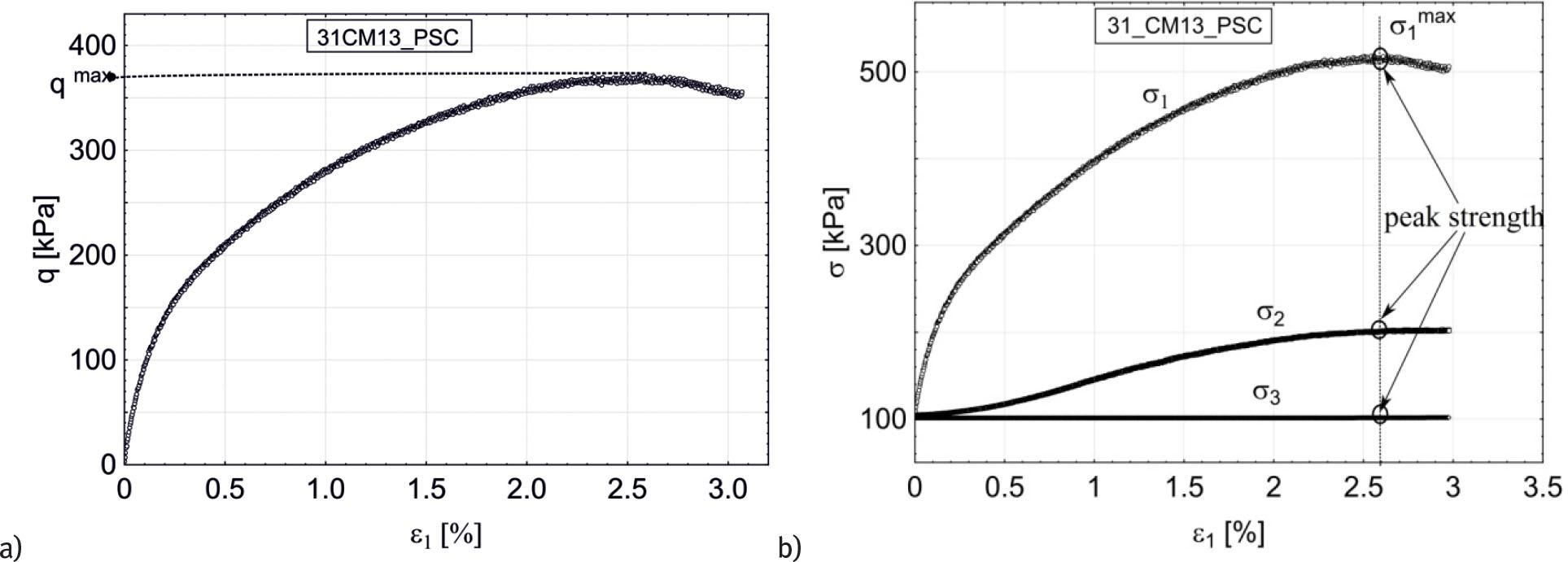The principal stress and axial strain curves for the selected test in plane strain conditions: a) q(ɛ1) and b) σ1(ɛ1), σ2(ɛ1) and σ3(ɛ1).

Unlike critical soil strength, peak strength depends on the initial density of soil. Most of the research on soil peak strength uses Mohr–Coulomb condition and so the dependence of peak friction angle on soil density. Been and Jefferies (1985, 1986) have shown the relationship between the peak friction angle and the soil state parameter, defined as the difference in void ratio between the initial and steady-state, at the same mean effective stress.

Bolton (1986) studied the relationship between the mobilized friction angle, critical state friction angle and soil relative density in plane strain conditions and proposed the equation describing this relationship. Chakraborty and Salgado (2010) confirmed Bolton's theory for low confining pressures (triaxial and plane strain tests). The effect of confining pressure on peak friction angle in the process of grain crushing is shown in Yamamuro and Lade (1996): as confining pressure increases, the peak friction angle decreases. Sadrekarimi and Olson (2011) or Sarkar et al. (2019) show, in turn, that there exists no clear relationship between the peak friction angle and the effective stress.

The direct dependence of the friction angle on the initial soil porosity was shown already in Lee (1970), where analysed the data obtained in drained tests by Bishop (1961) and Cornforth (1964). The tests were conducted at confining pressure of 275 kPa, both in axisymmetric and plane strain conditions.

Fig. 3 shows their results, completed by the data obtained in this study for Skarpa sand at similar confining pressures between 278 kPa and 295 kPa (Tables 2 and 4). The same tendency: a decrease in the internal friction angle with growing sample porosity is observed in true triaxial tests on Skarpa sand under plane strain conditions, but no quantitative agreement is found, because they are two different soils.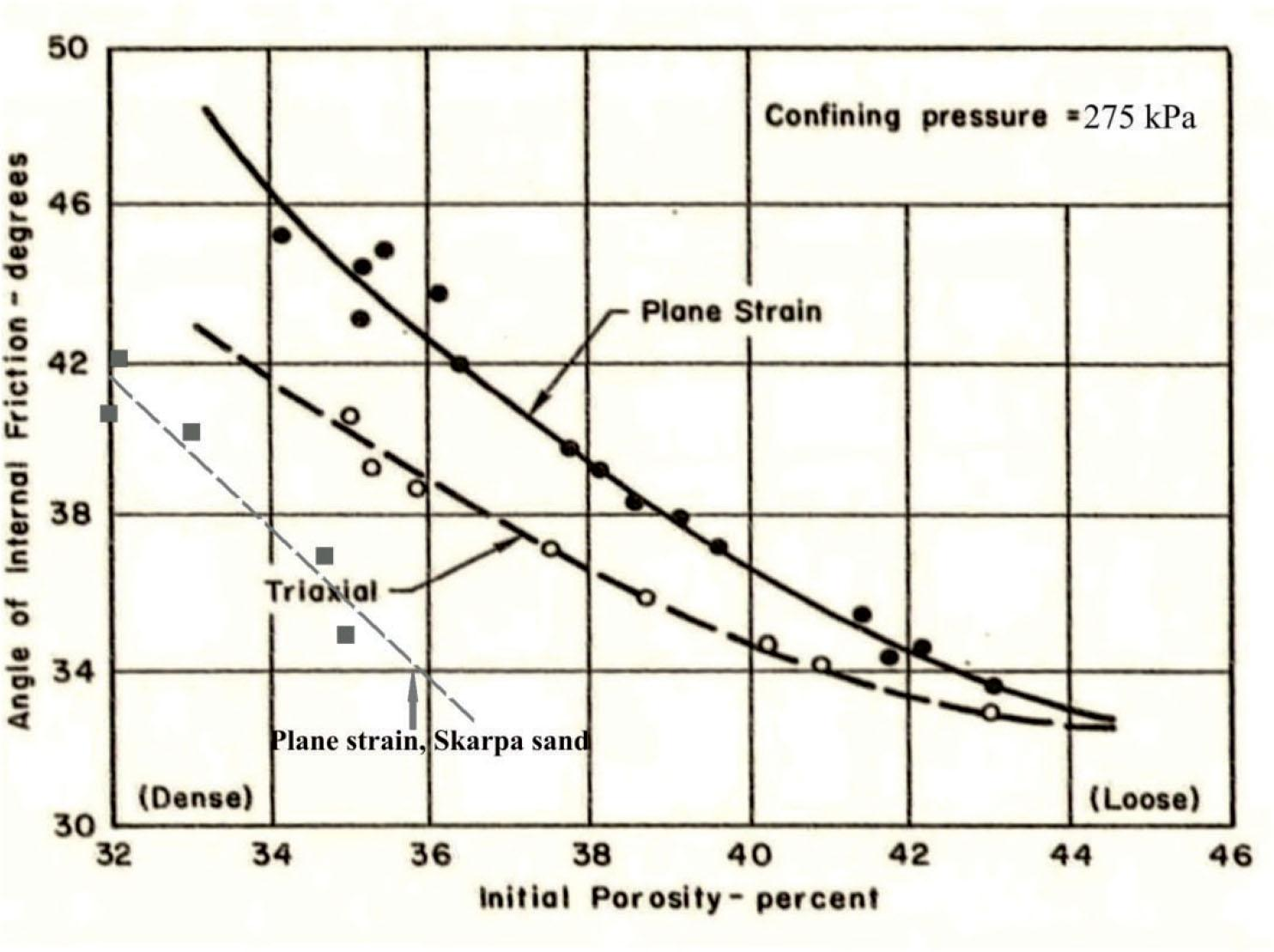Comparison of the results from drained triaxial and plane strain tests on sand  and true-triaxial tests on Skarpa sand.
Scope of the paper

The main purpose of this study is to establish, both experimentally and semi-theoretically, the parameters characterizing different soil failure criteria, presented in Section 2, and their relationship to the internal friction angle in a given range of initial soil densities in plane strain state.

The experimental way of finding the parameters involves determining the set of principal stress values σ1, σ2 and σ3, corresponding to the soil peak strength (Fig. 2). The same parameters are calculated semi-theoretically using the approach proposed by Vikash and Prashant (2010). The associated flow rule and plane strain condition are used to express the parameters as functions of the plane strain friction angle. The basic difference between the experimental and semi-theoretical approach concerns the intermediate stress σ2. In experiments, its value comes from the direct measurements; in calculations, it is determined on the base of the accepted assumptions and is different for each failure criterion. Validation of the Vikash and Prashant approach on the base of stress measurements is another purpose of the study.

Characteristics of soil failure criteria

The soil failure criteria are usually formulated using stress invariants, independent of the choice of the coordinate system. In standard triaxial conditions, two invariants of the stress tensor are enough to describe the state of stress and any load path in the stress space. In the true triaxial state, three different principal stresses exist; therefore, one more invariant is needed. Often, combinations of the basic invariants of the stress tensor are used to formulate failure criteria.

Soil failure state is graphically represented, in 3D stress space, by the surface which separates the allowable stress states from the states of uncontrolled plastic flow and is called yield surface. It is accepted in this study that the yield surface corresponds to the stress states at which a soil reaches its maximum (peak) strength. The failure criteria considered in this paper describe the shape of the yield surface and differ in cross-section on the deviatoric plane, perpendicular to the hydrostatic axis σ1 = σ2 = σ3 (Fig. 4).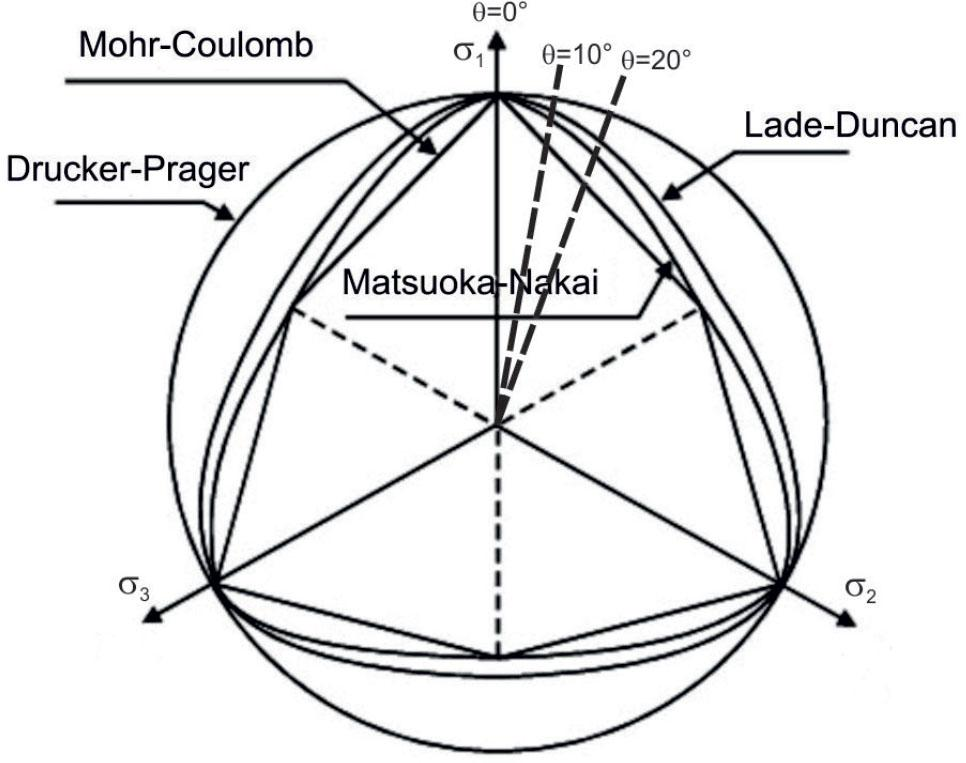Failure surfaces in the deviatoric plane, see Georgiadis et al. (2004). In plane strain conditions, Lode angle varies roughly from θ = 10° to θ = 20°.
Basic invariants of the stress tensor

The mean stress p and deviator stress q are most frequently employed for axisymmetric stress conditions. In the case of the true triaxial state, they are usually completed by Lode angle θ. In case of dry granular material invariants, p, q and θ can be expressed using the basic invariants of total or deviatoric stress tensor, defined by Eqs. (1)–(5):

The basic invariants of the total stress tensor σ: $I1=σii=σ1+σ2+σ3,$ {I_1} = {\sigma _{{\rm{ii}}}} = {\sigma _1} + {\sigma _2} + {\sigma _3}, $I2=12(σiiσjj−σijσij)=σ1σ2+σ1σ3+σ2σ3,$ {I_2} = {1 \over 2}\left( {{\sigma _{{\rm{ii}}}}{\sigma _{{\rm{jj}}}} - {\sigma _{{\rm{ij}}}}{\sigma _{{\rm{ij}}}}} \right) = {\sigma _1}{\sigma _2} + {\sigma _1}{\sigma _3} + {\sigma _2}{\sigma _3}, $I3=detσ=σ1σ2σ3,$ {I_3} = \det {\boldsymbol {\sigma }} = {\sigma _1}{\sigma _2}{\sigma _3},

The basic invariants of the deviatoric stress tensor $s=σ−13tr(σ)1$ {\boldsymbol {s}} = {\boldsymbol {\sigma }} - {1 \over 3}{\rm{tr}}\left( {\boldsymbol {\sigma }} \right){\bf{1}} : $J2=12s:s=12sijsij=16((σ1−σ2)2+(σ1−σ3)2+(σ2−σ3)2)$ {J_2} = {1 \over 2}{\boldsymbol {s:s}} = {1 \over 2}{s_{ij}}{s_{ij}} = {1 \over 6}\left( {{{\left( {{\sigma _1} - {\sigma _2}} \right)}^2} + {{\left( {{\sigma _1} - {\sigma _3}} \right)}^2} + {{\left( {{\sigma _2} - {\sigma _3}} \right)}^2}} \right) $J3=dets=13sijsjkski$ {J_3} = {\rm{det}}{\boldsymbol {s}} = {1 \over 3}{s_{ij}}{s_{jk}}{s_{ki}}

The mean stress p, deviator stress q and Lode angle θ are defined by Eqs. (6), (7) and (8).

$p=13(σ1+σ2+σ3)=3I1$ p = {1 \over 3}\left( {{\sigma _1} + {\sigma _2} + {\sigma _3}} \right) = 3{I_1} $q=12(σ1−σ2)2+(σ1−σ3)2+(σ2−σ3)2=3J2$ q = {1 \over {\sqrt 2 }}\sqrt {{{\left( {{\sigma _1} - {\sigma _2}} \right)}^2} + {{\left( {{\sigma _1} - {\sigma _3}} \right)}^2} + {{\left( {{\sigma _2} - {\sigma _3}} \right)}^2}} = \sqrt {3{J_2}} $θ=arccos(2σ1−σ2−σ3)2(σ1−σ2)2+(σ1−σ3)2+(σ2−σ3)2=13arccos(−33J32J23/2),$ \theta = \arccos {{\left( {2{\sigma _1} - {\sigma _2} - {\sigma _3}} \right)} \over {\sqrt 2 \sqrt {{{\left( {{\sigma _1} - {\sigma _2}} \right)}^2} + {{\left( {{\sigma _1} - {\sigma _3}} \right)}^2} + {{\left( {{\sigma _2} - {\sigma _3}} \right)}^2}} }} = {1 \over 3}\arccos \left( { - {{3\sqrt 3 {J_3}} \over {2{J_2}^{3/2}}}} \right),

In classical triaxial compression θ = 0°, in triaxial extension θ =60°, generally 0° < θ < 60°.

Lode angle is in some studies replaced by its alternative – the Bishop's parameter b is defined by Eq. (9) and related to θ by Eq. (10). $b=σ2−σ3σ1−σ3$ b = {{{\sigma _2} - {\sigma _3}} \over {{\sigma _1} - {\sigma _3}}} $b=12(1+3tg(θ−30°))$ b = {1 \over 2}\left( {1 + \sqrt 3 {\rm{tg}}\left( {\theta - 30^\circ } \right)} \right) An analysis of experimental studies shows that values of b under plane strain conditions lie within the range of 0.18–0.35 (assuming that 10° < θ < 20°) (Tatsuoka et al. 1986; Wanatowski and Chu 2007).

Failure criteria

Mohr–Coulomb (M-C) failure surface FM-C for non-cohesive soil can be described by equation (11): $FM-C=12(σ1−σ3)−12(σ1+σ3)sinφ=0,$ {F_{{\rm{M - C}}}} = {1 \over 2}\left( {{\sigma _1} - {\sigma _3}} \right) - {1 \over 2}\left( {{\sigma _1} + {\sigma _3}} \right)\sin \varphi = 0, where σ1 and σ3 are the maximum and the minimum principal stress and ϕM-C is the internal friction angle. On the deviatoric plane, the M-C criterion is represented by a hexagonal contour (Fig. 4) and ϕM-C is constant. FM-C does not depend on the intermediate stress σ2.

Drucker–Prager (D-P) failure criterion was established as a generalization of the Mohr–Coulomb condition; see Drucker and Prager (1952), and this criterion for non-cohesive soil is described by equation (12): $FD−P=J2−κD−PI1=0,$ {F_{{\rm{D}} - {\rm{P}}}} = \sqrt {{J_2}} - {\kappa _{{\rm{D}} - {\rm{P}}}}{I_1} = 0, where I1 and J2 are given by Eqs. (1) and (4) and κD-P is the specific soil parameter. On the deviatoric plane, the D-P is represented by a circle (Fig. 4).

Lade–Duncan (L-D) criterion – true triaxial experiments (especially in plane strain conditions) prove that shear strength for intermediate values of Lode angle (0° < θ < 60°) is higher than that resulting from the M-C criterion in the classical triaxial test; see Eekelen (1980), Lade and Duncan (1973) and Sławińska (2018). An empirical criterion which takes into account these observations is called Lade–Duncan (L-D) criterion (Eq. (13)): $FL−D=I13I3−κL−D=0,$ {F_{{\rm{L}} - {\rm{D}}}} = {{I_1^3} \over {{I_3}}} - {\kappa _{{\rm{L}} - {\rm{D}}}} = 0, where κL-D is the specific soil parameter and I1, I3 are defined by Eqs. (1) and (3).

Matsuoka–Nakai (M-N) criterion is proposed on the basis of spatially mobilized plane concept (Matsuoka and Nakai, 1974). This criterion, based on theoretical considerations, is described by the relation (14): $FM−N=I1I2I3−κM−N=0,$ {F_{{\rm{M}} - {\rm{N}}}} = {{{I_1}{I_2}} \over {{I_3}}} - {\kappa _{{\rm{M}} - {\rm{N}}}} = 0, where κM-N is the specific soil parameter and I1, I2, I3 are defined by Eqs. (1)–(3).

Failure criteria parameters in plane strain conditions

Soil parameters ϕ, κD-P, κL-D and κM-N, appearing in the failure criteria, can be determined directly from Eqs. (11)–(14) using the principal stress values corresponding to the peak soil strength measured experimentally or on the semi-theoretical way, where σ2 is determined assuming the associated flow rule and the plane strain condition and only σ1max and σ3 are measured.

Determination of failure criteria parameters from direct stress measurements

Transforming equations (11)–(14) gives the expressions (15)–(18) which allow to determine the parameters of failure criteria using the measured values of σ1max, σ2 and σ3: $κM−Cexp=arcsin(σ1−σ3σ1+σ3)=φps$ \kappa _{{\rm{M}} - {\rm{C}}}^{\exp } = \arcsin \left( {{{{\sigma _1} - {\sigma _3}} \over {{\sigma _1} + {\sigma _3}}}} \right) = {\varphi _{ps}} $κD−Pexp=J2I1$ \kappa _{{\rm{D}} - {\rm{P}}}^{\exp } = {{\sqrt {{J_2}} } \over {{I_1}}} $κM−Nexp=I1I2I3$ \kappa _{{\rm{M}} - {\rm{N}}}^{\exp } = {{{I_1}{I_2}} \over {{I_3}}} $κL−Dexp=I13I3$ \kappa _{{\rm{L}} - {\rm{D}}}^{\exp } = {{I_1^3} \over {{I_3}}}

Determination of failure criteria parameters by flow rule and plane strain condition

The associated flow rule comes from the rigorous formulation of the plasticity theory, while the non-associated one is only semi-theoretically postulated. The latter was introduced for soils to reduce the mismatch between measured and theoretically predicted volumetric strains in element tests. However, the solution of this particular problem introduces another problem, namely the non-coaxiality of the stress and strain tensors. It is difficult to measure experimentally and limits the application of the upper-bound theorem, based on the assumption of the associated flow rule and frequently used in limit analysis solutions of soil mechanics boundary value problems (e.g. Deusdado et al., 2016, di Santolo et al., 2012).

As a result, both the associated and non-associated flow rules are still used to model soil behaviour, depending on the nature of the problems studied.

Liu (2013) shows various results of triaxial compression tests on sands and their simulations using both the associated and non-associated flow rules. Similar simulations for more complex stress paths in the p - q - θ space can be found in Chan (1988) and Ling and Liu (2003). Their research demonstrates that the associated flow rule predicts well the results of triaxial compression tests for medium and medium-dense soils in the pre-peak stage and under drained conditions. The non-associated flow rule, on the other hand, gives better results for the unstable response of saturated loose sand and under undrained condition. According to these findings, it is justified to use the associated flow rule to analyse the behaviour of medium and medium-dense sand samples up to the peak strength, if volume changes are still significant and no distinct localization occurs.

Plastic flow rule can describe soil deformation at failure state. Its general formulation, as a non-associated flow rule, is given by Eq. (19): $ε˙ij=λ∂g∂σij$ {\dot \varepsilon _{ij}} = \lambda {{\partial g} \over {\partial {\sigma _{ij}}}} where $ε˙ij$ {\dot \varepsilon _{ij}} means the strain rate tensor, λ is the scalar coefficient and g is the plastic potential. The special case of the non-associated is the associated flow rule, where the plastic potential g coincides with the yield surface F (g = F). In such a case, the plastic potentials for D-P, M-N and L-D criteria are given by Eqs. (12)–(14), and it is possible to determine parameters κD-P, κL-D and κM-N theoretically by solving the set of equations (20). The set comes from the assumption of soil failure state (F = 0, where F means FD-P, FL-D or FM-N) and the plane strain condition 2 = 0, which substituted to the flow rule (19) gives $∂F∂σ2=0$ {{\partial F} \over {\partial {\sigma _2}}} = 0 . Such way of calculating parameters κD-P, κL-D and κM-N was proposed by Vikash and Prashant (2010). ${ F=0dε2=∂F∂σ2=0$ \left\{ {\matrix{ {F = 0} \hfill \cr {d{\varepsilon _2} = {{\partial F} \over {\partial {\sigma _2}}} = 0} \hfill \cr } } \right.

In the Appendix, the set (20) is presented in the expanded form, specific for each failure condition. The set can be completed by Eq. (21), valid in plane strain conditions, to express κD-P, κL-D and κM-N as functions of the internal friction angle ϕps. $σ1σ3=1+sinφps1−sinφps$ {{{\sigma _1}} \over {{\sigma _3}}} = {{1 + \sin {\varphi _{{\rm{ps}}}}} \over {1 - \sin {\varphi _{{\rm{ps}}}}}}

To solve (20), first the intermediate principal stress σ2 is determined as a function of σ1 and σ3, separately for D-P, L-D and M-N yield conditions: $σ2D−P=σ32+σ12σ1+σ3$ \sigma _2^{{\rm{D}} - {\rm{P}}} = {{\sigma _3^2 + \sigma _1^2} \over {{\sigma _1} + {\sigma _3}}} $σ2L−D=σ1+σ32$ \sigma _2^{{\rm{L}} - {\rm{D}}} = {{{\sigma _1} + {\sigma _3}} \over 2} $σ2M−N=σ1σ3$ \sigma _2^{{\rm{M}} - {\rm{N}}} = \sqrt {{\sigma _1}{\sigma _3}}

It can be seen from Eqs. (22)–(24) that each condition gives the different expression for the intermediate stress σ2. Finally, the following expressions for κD-P, κL-D and κM-N are obtained from the set (20), taking into account Eqs. (21)–(24): $κD−P=σ1−σ323σ32+σ1σ3+σ12=sinφps33+sin2φps$ {\kappa _{{\rm{D}} - {\rm{P}}}} = {{{\sigma _1} - {\sigma _3}} \over {2\sqrt 3 \sqrt {\sigma _3^2 + {\sigma _1}{\sigma _3} + \sigma _1^2} }} = {{\sin {\varphi _{{\rm{ps}}}}} \over {\sqrt 3 \sqrt {3 + {{\sin }^2}{\varphi _{{\rm{ps}}}}} }} $κL−D=27(σ32+2σ1σ3+σ12)4σ1σ3=274−sin2φps$ {\kappa _{{\rm{L}} - {\rm{D}}}} = {{27\left( {\sigma _3^2 + 2{\sigma _1}{\sigma _3} + \sigma _1^2} \right)} \over {4{\sigma _1}{\sigma _3}}} = {{27} \over {4 - {{\sin }^2}{\varphi _{ps}}}} $κM−N=σ32+3σ1σ3+σ12+22σ12σ32+2σ13σ3+σ1σ33σ1σ3=5−sin2φpscos2φps+4cosφps$ {\kappa _{{\rm{M}} - {\rm{N}}}} = {{\sigma _3^2 + 3{\sigma _1}{\sigma _3} + \sigma _1^2 + 2\sqrt {2\sigma _1^2\sigma _3^2 + 2\sigma _1^3{\sigma _3} + {\sigma _1}\sigma _3^3} } \over {{\sigma _1}{\sigma _3}}} = {{5 - {sin^2}{\varphi _{ps}}} \over {{cos^2}{\varphi _{ps}}}} + {4 \over {{cos}{\varphi _{ps}}}}

True triaxial tests in plane strain conditions

The series of tests in true triaxial apparatus in plane strain conditions were performed to verify both approaches of determining failure criteria parameters. Limiting the strains to plane (two-dimensional) case induces some partly controlled three-dimensional stress state, where σ2 comes from the soil reaction and cannot be applied a priori, but has to be measured. The non-zero σ2 in plane strain condition has always been a problem in interpreting the results of standard 2D soil mechanics tests.

The tests in this study were carried out on Skarpa sand samples at different confining pressures and initial void ratios. All the tests were performed in dry conditions. Basic properties of Skarpa sand are collected in Table 1.

Parameters of Skarpa sand.

 Specific density [kg/m3] 2650 Mean particle size [mm] D50 - 0.42 Uniformity coefficient [ − ] U = 2.5 Minimum void ratio [ − ] emin = 0.432 Maximum void ratio [ − ] emax = 0.677
Test procedure

The plane strain tests, with deformation fixed in x2 principal stress direction (ɛ2 = 0, Fig. 5), were carried out in true triaxial apparatus. The Electro-Mechanical True Triaxial Apparatus (EMTTA) is manufactured by the British company GDS Instruments Ltd. and is shown in Fig. 6. Plane strain condition can be imposed on the rectangular samples of 150 × 75 × 75 mm dimensions in this type of true triaxial apparatus by fixing the position of the side platens to prevent their movement (Fig. 7). Depending on the way the stresses are exerted on the test specimen, EMTTA is mixed type, with flexible membrane (horizontal stress) and rigid end platens (axial stress).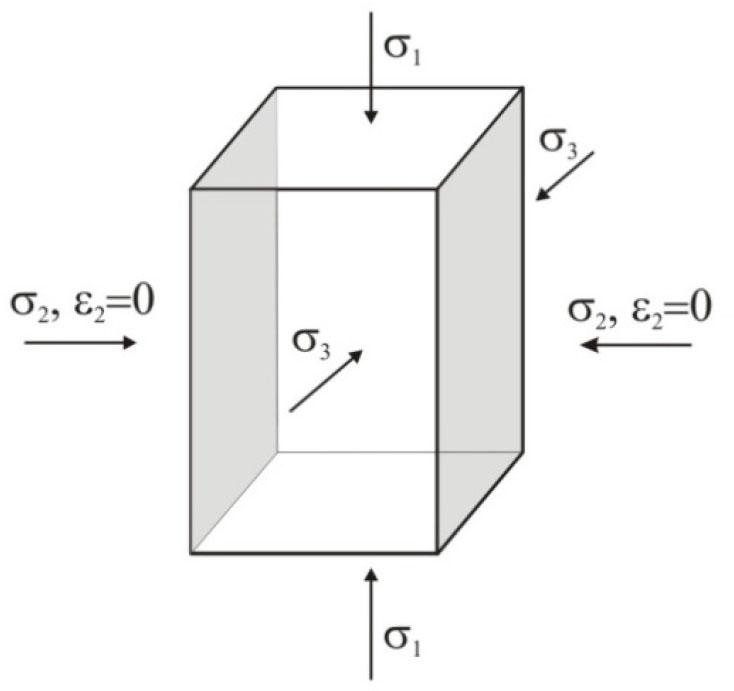Layout of the soil sample under plane strain conditions in EMTTA.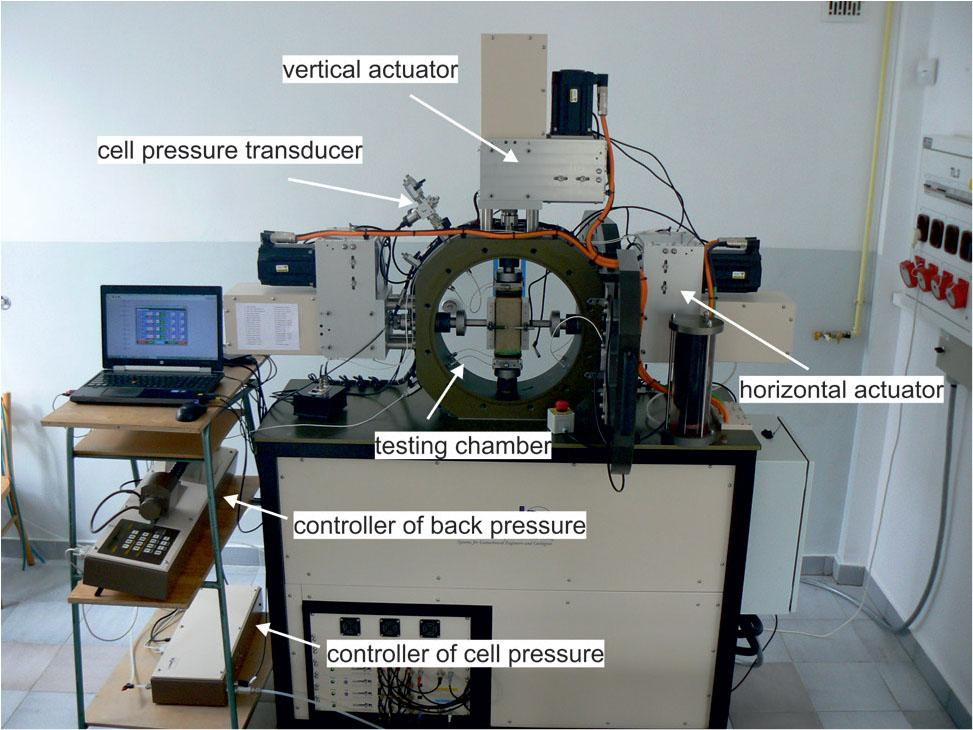Components of EMTTA, used in the study.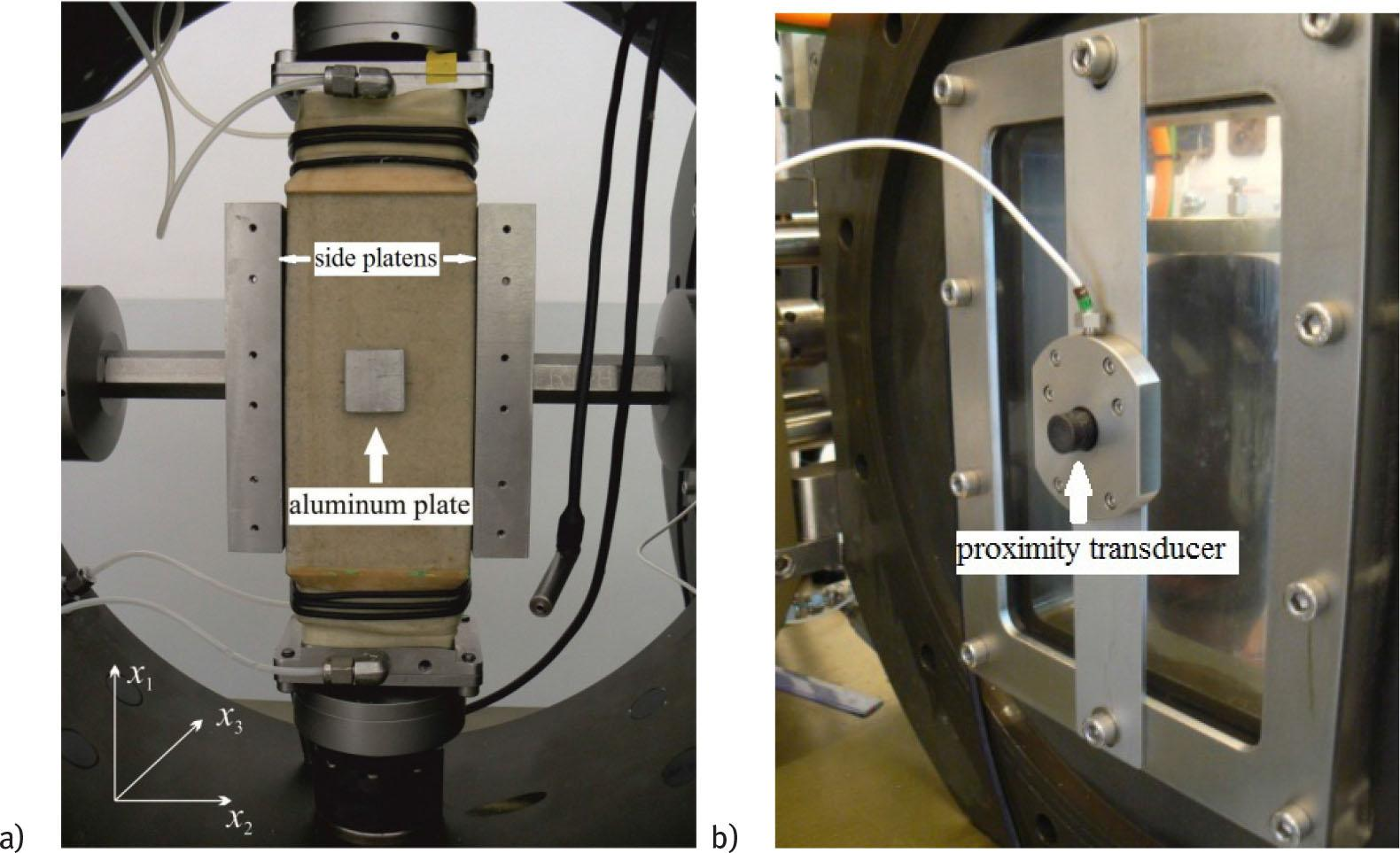a) The GDS EMTTA chamber with a sample prepared for the test. The role of the side plates is to prevent soil deformations in the x2direction, b) proximity transducer on the doors of the measurement cell (test chamber).

Fig. 7a shows the sample ready for the test, with the side platens fixed. After installing the sample in the testing chamber (measurement cell, Fig. 6), its doors are closed and it is filled with water, then the side platens are gently pressed against the specimen until the difference between horizontal stresses σ2 - σ3 is about 2–3 kPa, to secure the proper contact. To reduce friction between the membrane and the side plates, the plates are lubricated with a special lubricant.

Specimen base pedestal is connected to the pressure/volume controller (back pressure controller, Fig. 6), which is used to apply and measure the pore water pressure and volume changes. Cell pressure is controlled by a pneumatic-hydraulic system (a cell pressure controller), where required value can be set. In addition, TTA is also equipped with a cell pressure transducer (see Fig. 6) located inside the chamber.

Vertical actuators shown in Fig. 6 are used to apply the major σ1 principal stress. The horizontal stress σ3 is applied through the water pressure in the chamber, and σ2 is recorded by a gauge located on vertical actuators.

In order to measure the specimen displacements in x3 direction, two proximity transducers are installed on the inner side of both cell doors, exactly at their centre (Fig. 7b). When the sample deforms, the changes in a distance between the square aluminium plate (Fig. 7a), adhered to the sample and the transducers, are recorded, giving the local value of the displacement. Based on this measurement, ɛ3 is calculated. Displacement in x1 direction is measured by LVDT transducers attached to each actuator; the vertical strain ɛ1 is calculated on its base.

Soil samples are prepared in a membrane-lined split mould by air pluviation. This method involves preparing a soil sample using a funnel with a nozzle of approximately 5 mm. The weighed sand is placed in the funnel at the selected distance from the centre of the mould. The height of the funnel and the mass of sand are determined by ‘trial and error’ to obtain the appropriate relative density (Li et al., 2018).

Eleven tests were carried out according to the same procedure. The tests consisted of two phases:

Phase 1 (isotropic compression): the sample is loaded isotropically by increasing water pressure in the testing chamber (σ3c in Table 2). This is not carried out under plane strain conditions. The side plates, pressed against the sample, move along with it, while a constant set value of lateral stress (about 2–3 kPa) is maintained.

Phase 2 (shear): the sample is vertically loaded with a constant vertical displacement rate of 15 mm/hour at constant chamber pressure (σ1 = σ3c = const) in plane strain conditions.

The samples’ porosities were between 0.316 and 0.36 (the relative densities $IDc$ I_D^c after isotropic compression between 0.465 and 0.878), and thus medium-dense, dense and very dense samples were tested. The applied confining pressures were in a range of 50–400 kPa.

Measurement results

Table 2 contains the initial conditions of all tested samples: the initial void ratio at the start of Phase 1 (e), the void ratio and porosity after isotropic compression, at the beginning of Phase 2 (ec, nc) and the applied confining pressure (σ3c). Also, the relative density ID, defined by Eq. (28), is given. $ID=emax−eemax−emin$ {I_D} = {{{e_{{max}}} - e} \over {{e_{{max}}} - {e_{{min}}}}} where emax and emin are maximum and minimum void ratios determined in the Proctor test. The porosity nc is calculated on the base of a void ratio, using Eq. (29): $n=ee+1$ n = {e \over {e + 1}}

The basic results of the eleven tests listed in Table 1 are gathered in Figs 8–11. Each of the figures shows the full set of curves for all the tests: deviator stress (Fig. 8), maximum principal stress (Fig. 9), principal stress in the direction of fixed strain (Fig. 10) and volumetric strain (Fig. 11), as functions of the axial strain.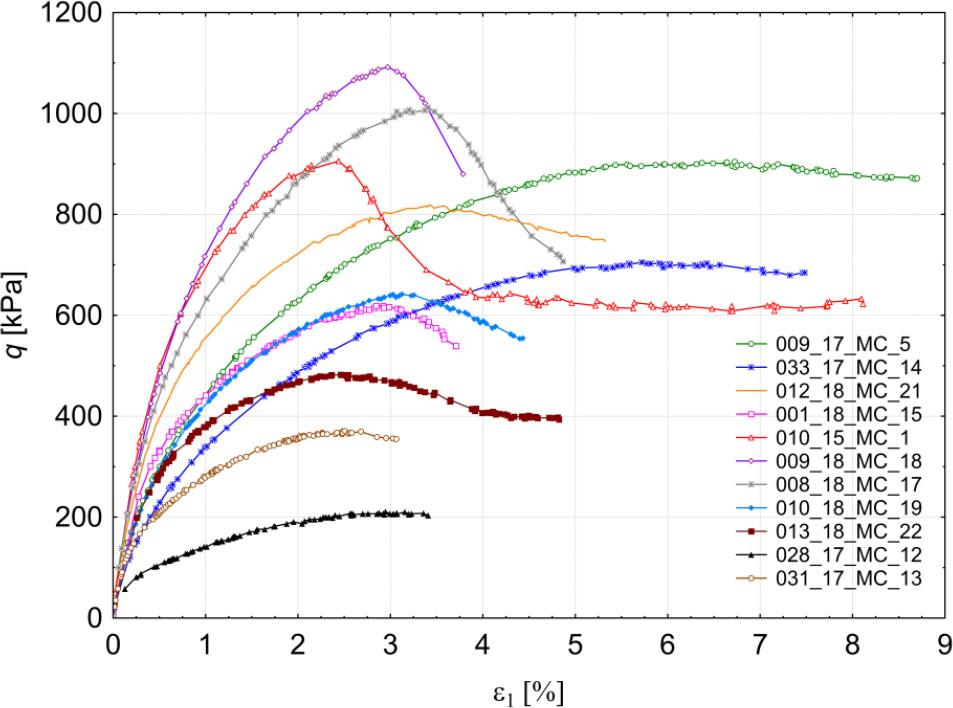Results of the experimental tests listed in Table 1: deviator stress as a function of the axial strain q(ɛ1).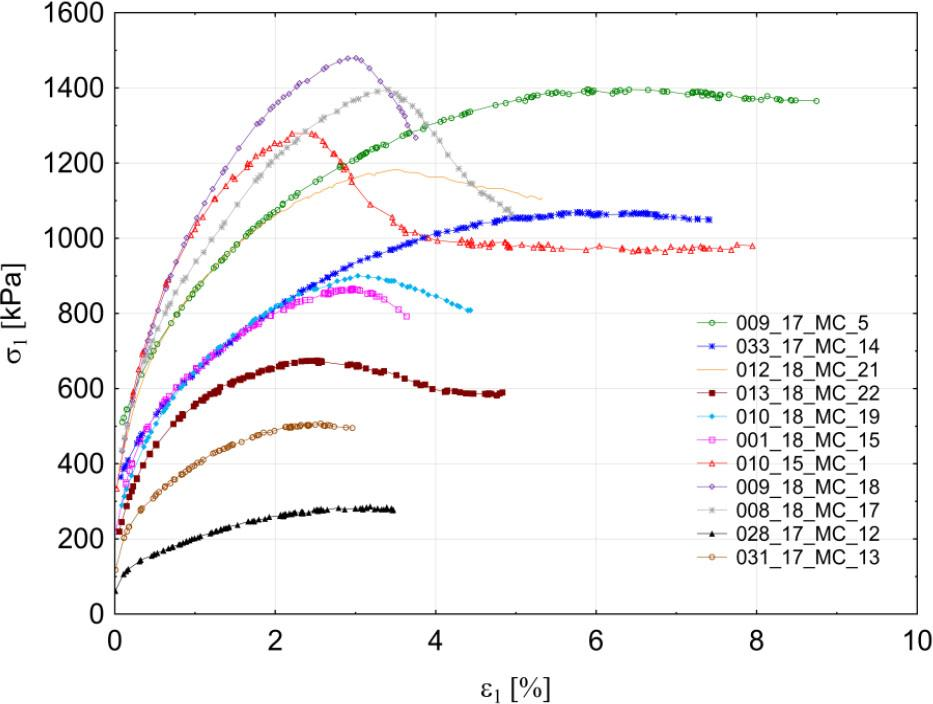Results of the experimental tests listed in Table 1: maximum principal stress as a function of the axial strain σ1(ɛ1).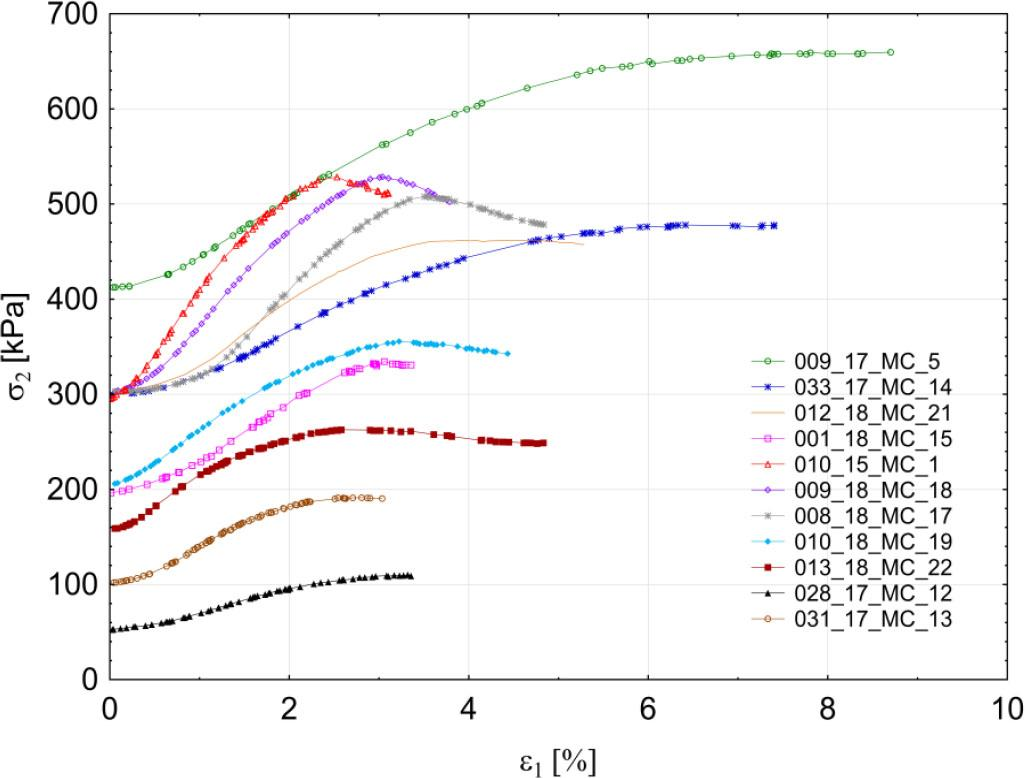Results of the experimental tests listed in Table 1: principal stress in the direction of fixed strain (ɛ2 = 0) as a function of the axial strain σ2(ɛ1).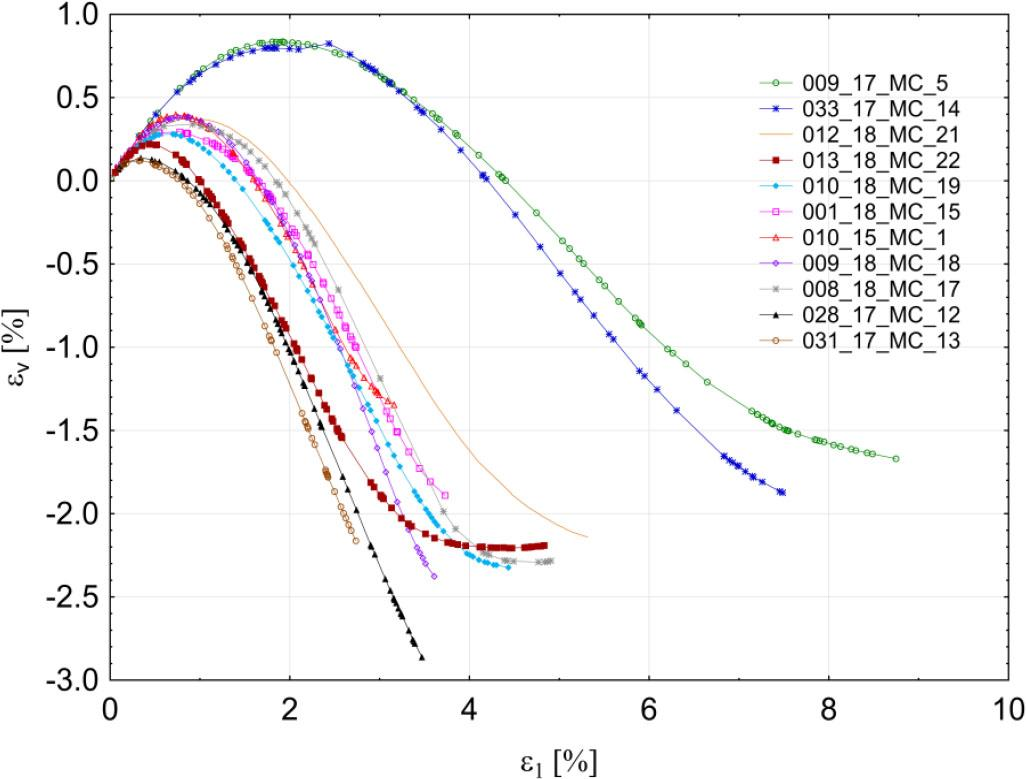Results of the experimental tests listed in Table 1: volumetric strain as a function of the axial strain ɛv(ɛ1).

The sets are highly varied because they correspond to the samples of different densities (medium to very dense), tested at different confining pressures (Table 2). The axial strain at which the peak strength occurs is determined for each test on the base of Fig. 8, and then, the corresponding values of σ1max, σ2 and σ3 are established, like it is demonstrated in Fig. 2.

Initial test conditions.

Test e ID σc3[kPa] ec $IDc$ {\boldsymbol {I}}_{\boldsymbol {D}}^{\boldsymbol {c}} nc
009_17_MC_5 0.585 0.376 391 0.563 0.465 0.36
033_17_MC_14 0.559 0.482 293 0.548 0.527 0.354
012_18_MC_21 0.541 0.555 292 0.532 0.592 0.347
013_18_MC_22 0.519 0.645 146 0.514 0.665 0.339
010_18_MC_19 0.517 0.653 195 0.508 0.690 0.337
001_18_MC_15 0.521 0.637 191 0.499 0.727 0.333
010_15_MC_1 0.496 0.739 278 0.490 0.763 0.329
009_18_MC_18 0.488 0.771 292 0.480 0.804 0.324
008_18_MC_17 0.489 0.767 295 0.476 0.820 0.322
028_17_MC_12 0.467 0.857 52 0.462 0.878 0.316
031_17_MC_13 0.469 0.849 99 0.462 0.878 0.316

Fig. 11 shows that dilative behaviour is observed in all the tested samples. The volumetric strain reaches the maximum (compression is positive), which is typical for dense samples. The maximum value of the deviator stress q was observed in test ‘009_17_MC_5’ (Fig. 8). The corresponding axial stress equals σ1max = 1402 kPa (Fig. 9). At this range of stress, no grain crushing is possible in case of silica sand, so it is accepted that no grain crushing occurred in the tests; see Yang et al. (2010). Table 3 presents the measured values of σ1max, σ2, σ3, defining the peak strength of each sample, and p, q, θ and b, calculated on their base.

Characteristics of peak strength state for the tested samples.

Test σ1max [kPa] σ2 [kPa] σ3 [kPa] p [kPa] q [kPa] b [ − ] θ [ ° ]
009_17_MC_5 1402 653 391 815 909 0.26 14.46
033_17_MC_14 1072 479 293 615 705 0.24 13.21
012_18_MC_21 1184 459 292 645 821 0.19 10.14
013_18_MC_22 678 262 146 362 485 0.22 11.97
010_18_MC_19 902 355 195 484 642 0.23 12.46
001_18_MC_15 870 332 191 464 621 0.21 11.35
010_15_MC_1 1291 529 278 699 914 0.25 13.76
009_18_MC_18 1483 528 292 768 1092 0.20 10.78
008_18_MC_17 1396 506 295 732 1012 0.19 10.40
028_17_MC_12 287 109 52 149 212 0.24 13.44
031_17_MC_13 508 191 99 266 372 0.22 12.38

Figs 8–11 represent the whole course of the experimental tests, showing pre- and post-peak behaviour. Only the pre-peak part of the tests, defined on the basis of Figs 8–11, is analysed in the paper. Before the onset of localization, the deviator peak strength (not the critical strength) is used. The literature shows that localization is observed after the deviator peak strength has been reached, e.g. Leśniewska et al (2012) and Desrues and Viggiani (2004).

Fig. 12 shows the relationship between principal stress components, corresponding to the peak soil strength q: σ1max3) and σ23), which can be estimated with sufficient accuracy by straight lines (r = 0.94 and r = 0.9, where r is Pearson's coefficient). Fig. 12 also confirms that in all the tests, the measured σ2 is always the intermediate principal stress.

Fig. 13 collects the values of Lode angle calculated using Eq. (8) and the data from Table 3, and it suggests that there is no statistically significant difference in Lode angle due to varying principal stress σ2 or confining pressure σ3. The Lode angle is considered constant at θ = 12.6° (calculated as an arithmetic average), but its scatter around this value is considerable, which is probably related to the unavoidable problem of forming soil samples of repeatable structure. The value of the Lode angle may also depend in a more complex way on the initial density of the soil sample or confining pressure. This problem is open.

The constant and relatively low value of Lode angle obtained for all the tests from Table 3 confirms that in plane strain conditions, the influence of the intermediate stress σ2 on the soil peak behaviour is limited and does not depend on the confining pressure. The question remains, how to estimate the magnitude of this influence.

Values of parameters $κM−Cexp(φps)$ \kappa _{{\rm{M}} - {\rm{C}}}^{\exp }\left( {{\varphi _{ps}}} \right) , $κD−Pexp$ \kappa _{{\rm{D}} - {\rm{P}}}^{\exp } , $κM−Nexp$ \kappa _{{\rm{M}} - {\rm{N}}}^{\exp } and $κL−Dexp$ \kappa _{{\rm{L}} - {\rm{D}}}^{\exp } , obtained from Eqs. (15)–(18) by substituting σ1max, σ2 and σ3, which correspond to the peak strength of the tested samples, are collected in Table 4, column ‘A’. Values of parameters κD-P, κL-D and κM-N, calculated from Eqs. (25)–(27) by substituting σ1max and σ3, are given in Table 4, column ‘B’.

Characteristic parameters of Drucker–Prager, Matsuoka–Nakai and Lade–Duncan soil failure criteria, obtained from direct stress measurements (A) and the associated flow rule assuming plane strain conditions (B).

Test A. Direct stress measurements Eqs. (15)–(18) B. Flow rule and plane strain condition Eqs. (25)–(27)

ϕps $κD−Pexp$ \kappa _{{\rm{D}} - {\rm{P}}}^{\exp } $κM−Nexp$ \kappa _{{\rm{M}} - {\rm{N}}}^{\exp } $κL−Dexp$ \kappa _{{\rm{L}} - {\rm{D}}}^{\exp } $σ2D-P$ \sigma _2^{{\rm{D - P}}} $σ2M-N$ \sigma _2^{{\rm{M - N}}} $σ2L-D$ \sigma _2^{{\rm{L - D}}} $κD-Pflow rule$ \kappa _{D{\rm{ - }}P}^{f{\rm{low}}\,{\rm{rule}}} $κM-Nflow rule$ \kappa _{M{\rm{ - }}N}^{f{\rm{low}}\,{\rm{rule}}} $κL-Dflow rule$ \kappa _{L{\rm{ - }}D}^{f{\rm{low}}\,{\rm{rule}}}
009_17_MC_5 34.3° 0.21 11.7 40.9 1181.5 740.4 896.5 0.179 11.7 39.6
033_17_MC_14 34.8° 0.22 11.9 41.7 904.8 560.4 682.5 0.181 11.8 40.0
012_18_MC_21 37.2° 0.25 12.5 45.7 1007.5 588.0 738 0.190 12.3 42.5
013_18_MC_22 40.2° 0.26 13.2 49.4 583.8 314.6 412 0.202 13.1 46.3
010_18_MC_19 40.1° 0.26 13.1 49.0 776.3 419.3 548.5 0.201 13.1 46.2
001_18_MC_15 39.8° 0.26 13.1 49.0 747.8 407.6 530.5 0.200 13.0 45.7
010_15_MC_1 40.2° 0.25 13.1 48.6 1111.5 599.1 784.5 0.202 13.1 46.3
009_18_MC_18 42.1° 0.27 13.8 53.4 1287.1 658.1 887.5 0.209 13.7 49.1
008_18_MC_17 40.6° 0.27 13.4 50.9 1203.9 641.7 745.5 0.203 13.2 46.9
028_17_MC_12 43.9° 0.27 14.3 55.3 251.0 122.1 169.5 0.215 14.3 52.0
031_17_MC_13 42.4° 0.27 13.8 52.9 441.3 224.3 303.5 0.209 13.7 46.5
Discussion of results

The data included in Table 4 are presented in Figs 14–17. First, the relationship between the intermediate principal stress σ2 for Drucker–Prager, Matsuoka–Nakai and Lade–Duncan criteria, obtained from Eqs. (22)–(24) and measured in the experiments, is analysed, and then Vikash and Prashant solution, given by Eqs. (25)–(27), is verified experimentally.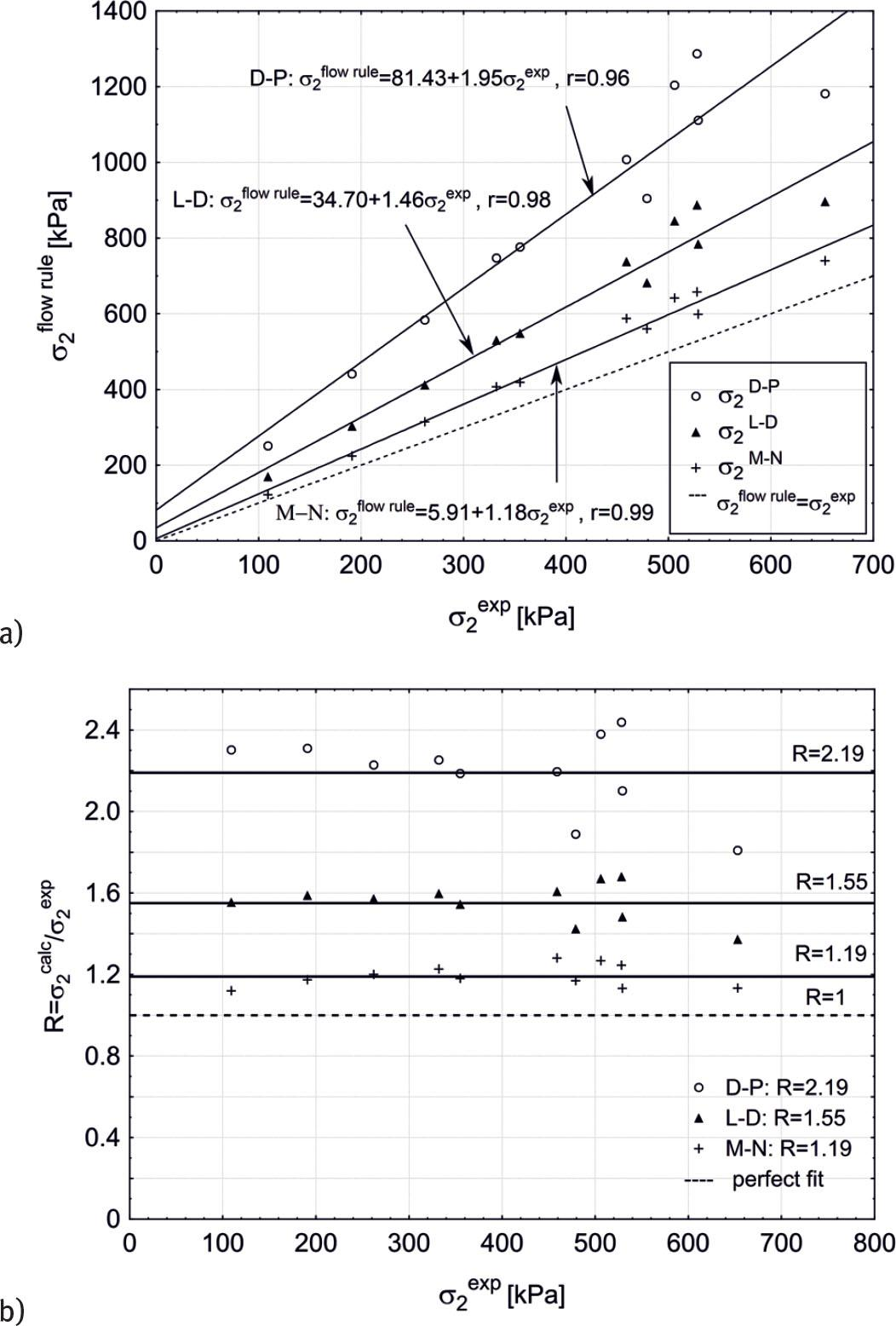The intermediate stress σ2, obtained for Drucker–Prager (D-P), Matsuoka–Nakai (M-N) and Lade–Duncan (L-D) failure criteria, assuming plane strain condition and the associated flow rule, as function of the measured σ2 (Table 3): (a) σ2calc (σ2exp) and (b) R(σ2exp), where R = σ2calc/σ2exp.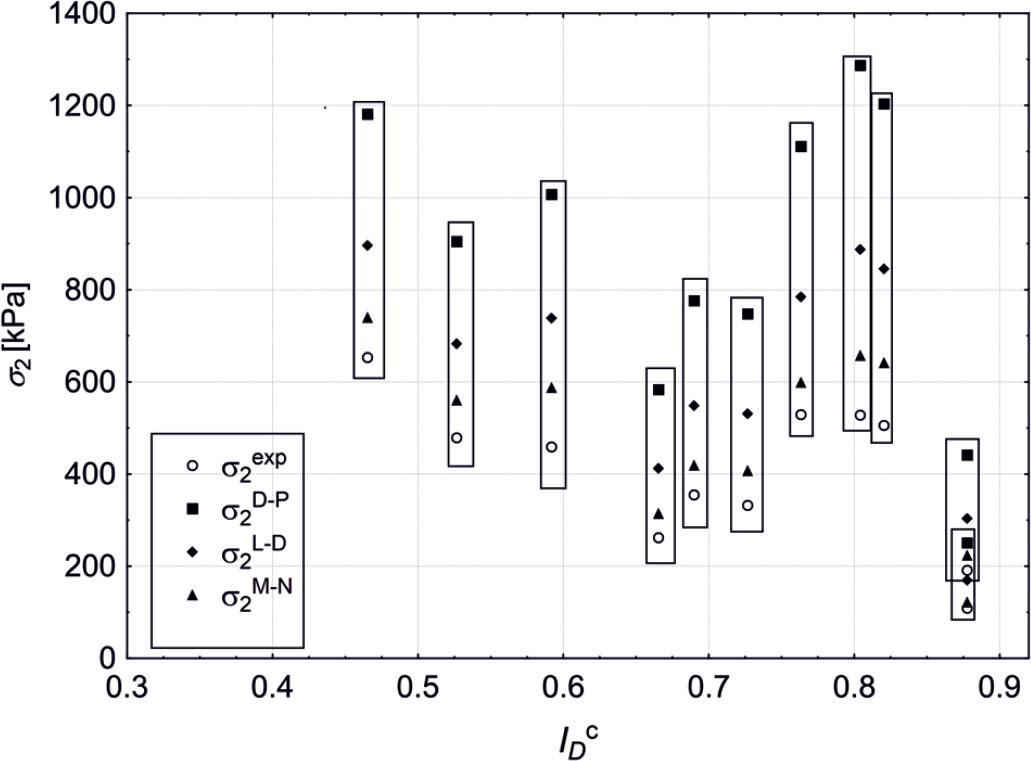Dependence of the intermediate stress σ2 (Tables 3 and 4) on the initial relative density of Skarpa sand.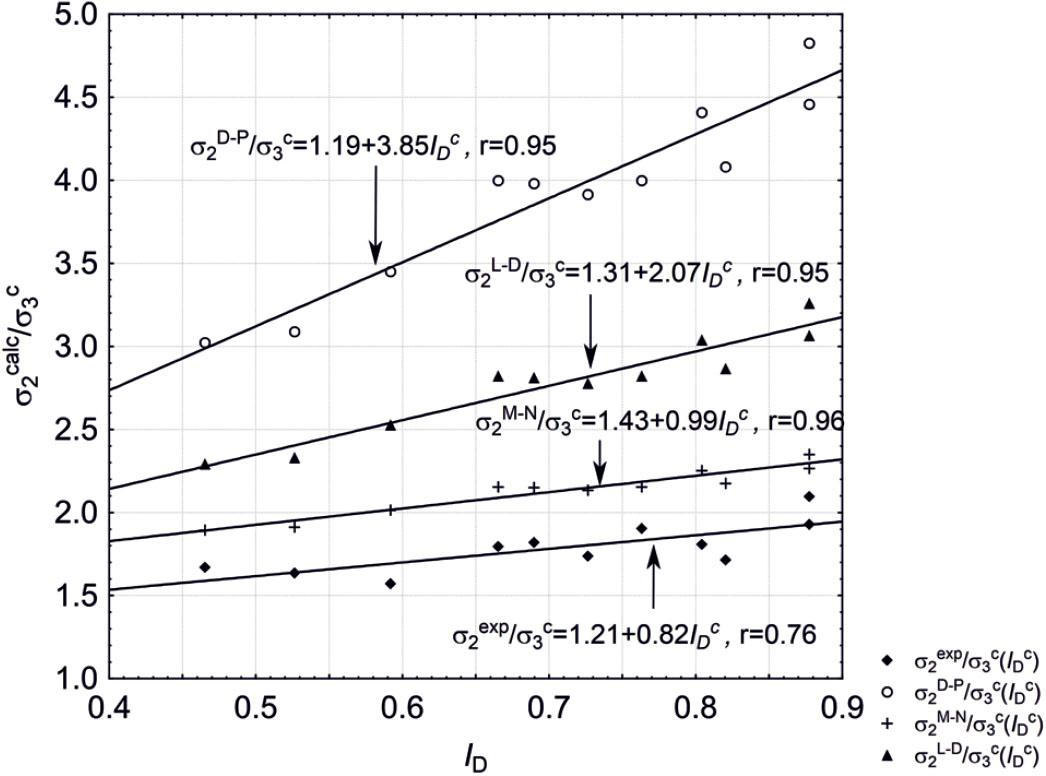Dependence of the ratio of intermediate stress σ2 to confining pressure σ3 (Table 4) on the initial relative density of Skarpa sand.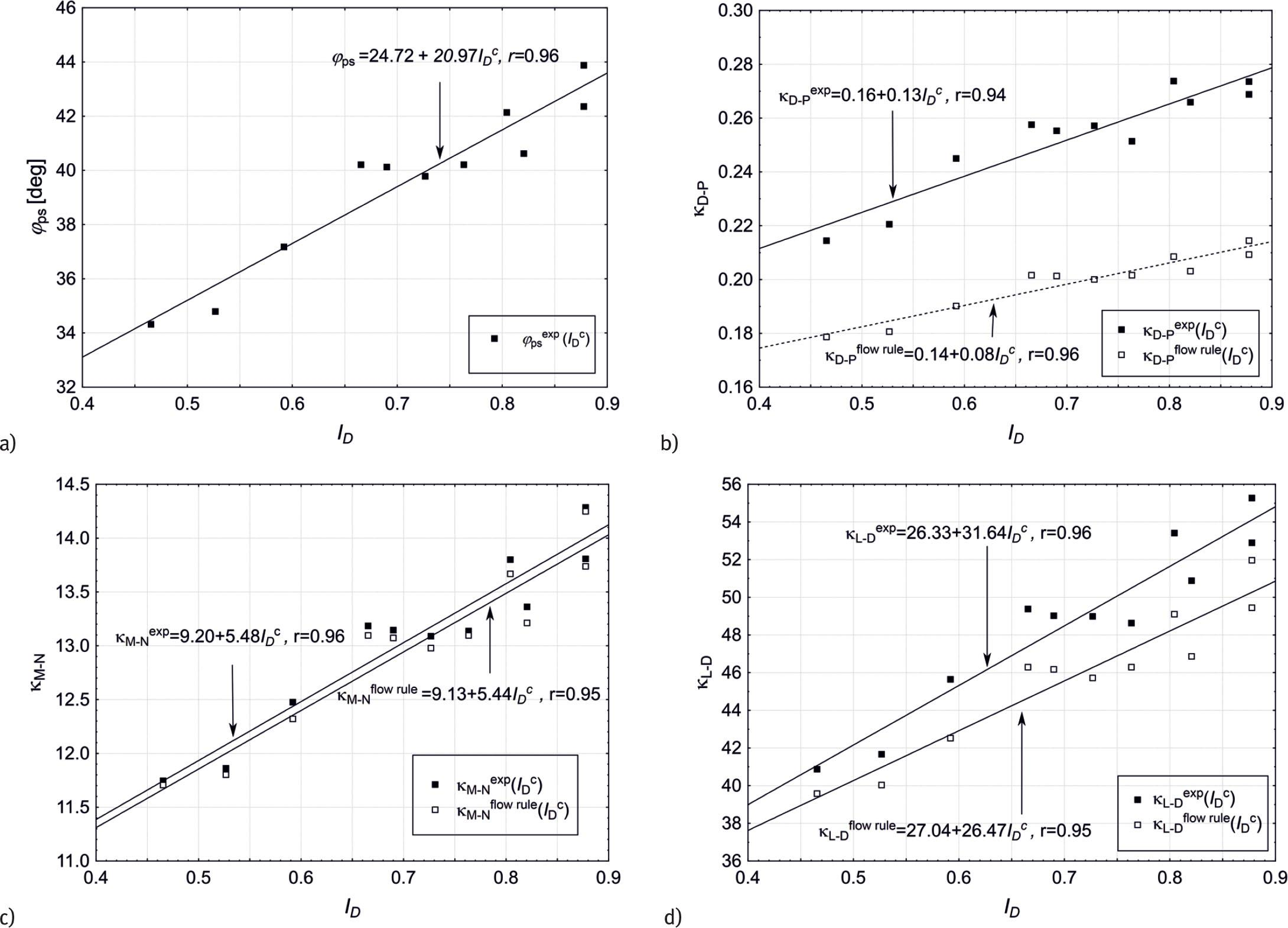Parameters of Mohr–Coulomb (M-C), Drucker–Prager (D-C), Matsuoka–Nakai (M-N) and Lade–Duncan (L-D) failure criteria depending on soil relative density: (a) friction angle ϕ, (b)–(d) comparison of κD-P, κL-D and κM-N, obtained by Eqs. (16)–(18) (full stress state measurement) and Eqs. (25)–(27) (plane strain condition – Vikash and Prashant approach).
Intermediate stress σ2

Fig. 14a presents σ2D-P, σ2M-N and σ2L-D as functions of the experimental σ2, taken from Table 3. A perfect fit between the calculated and measured values would mean that they lie on the dashed line σ2calc = σ2exp, shown in the figure.

A linear relationship between the calculated and measured intermediate stress σ2 for all three selected criteria is found, but only Matsuoka–Nakai is close to the perfect fit. The ratio R = σ2calc / σ2exp plotted in Fig. 14b shows three constant trends: 2.15 for Drucker–Prager, 1.52 for Lade–Duncan and 1.17 for Matsuoka–Nakai condition, with perfect fit equal to 1. It means that the Vikash and Prashant (2010) approach, highly overestimates the influence of the intermediate stress in plane strain conditions for Drucker–Prager, gives about 50% overestimation for Lade–Duncan and is close to measured values for Matsuoka–Nakai criterion.

Fig. 15 shows the dependence of the intermediate stress σ2, measured and calculated by Eqs. (25)–(27), on the initial relative density of Skarpa sand. There is no clear tendency visible because the tests presented in the paper were performed at different confining pressures. If the ratio of σ2 to the confining pressure σ3c is examined instead (Fig. 16), the linear trends appear both in the case of measured and calculated values and again the Matsuoka–Nakai criterion is closest to reality.

Experimental validation of Vikash and Prashant approach

Parameters ϕps, κD-, κL-D and κM-N, obtained by Eqs. (15)–(18), requiring knowledge of the full set of principal stresses σ1, σ2 and σ3, and by Vikash and Prashant approach, which requires measurement of two principal stresses only (σ1 and σ1), are shown in Fig. 17, as functions of the relative density ID, for all the true triaxial tests (Tables 2–4).

It is possible in Vikash and Prashant approach to replace σ1 and σ3 by the internal friction angle ϕps (Eqs. (25)–(27)). The internal friction angle for plane strain condition can be obtained directly from the relation (21) and play the role of the independent variable that specifies κD-P, κL-D and κM-N.

Fig. 17(a) shows the relation between the M-C friction angle ϕps and the relative density $IDc$ I_D^c of Skarpa sand, which is linear within the given density range, thus allowing for the equivalent presentation of κD-P, κL-D and κM-N as functions of ϕps or $IDc$ I_D^c independent variables. $IDc$ I_D^c was selected for Fig. 17(b)–(d). The figure confirms the previous finding that the Vikash and Prashant approach gives a very good result for the Matsuoka–Nakai failure criterion – the κM-N values obtained in this case by the two approaches almost coincide in Fig. 17c.

There is no such a good fit in case of Drucker–Prager and Lade–Duncan conditions. As Matsuoka–Nakai criterion is most commonly used to estimate soil strength in complex stress states, this finding can help to determine parameters necessary for numerical analysis of plane strain problems in a relatively simple way.

All three failure conditions give linear increase of κM-N, κD-P and κM-N with soil relative density, regardless of the way they are obtained, as expected.

The linear fits for the failure criteria parameter κexp, obtained from the direct stress measurement of σ1max, σ2 and σ3 (Table 3) and expressed as a function of the relative soil density (ID) or the plane strain friction angle ϕps, are given in Tables 5 and 6, respectively.

The linear fits $κexp(IDc)$ {\kappa ^{\exp }}\left( {I_D^c} \right) and the corresponding Pearson's correlation coefficients.

Linear fit Pearson's coefficient r
Drucker–Prager $κD−Pexp=0.16IDc+0.13$ \kappa _{{\rm{D}} - {\rm{P}}}^{\exp } = 0.16I_D^c + 0.13 rD-P = 0.94
Lade–Duncan $κL−Dexp=26.33IDc+31.64$ \kappa _{{\rm{L}} - {\rm{D}}}^{\exp } = 26.33I_D^c + 31.64 rL-D = 0.96
Matsuoka–Nakai $κM−Nexp=9.20IDc+5.48$ \kappa _{{\rm{M}} - {\rm{N}}}^{\exp } = 9.20I_D^c + 5.48 rM-N = 0.96

The linear fits κexp(ϕps)and the corresponding statistics Pearson's correlation coefficients.

Linear fit Pearson's coefficient r
Drucker–Prager $κD−Pexp=0.006353 φps+0.001497$ \kappa _{{\rm{D}} - {\rm{P}}}^{\exp } = 0.006353\,{\varphi _{{\rm{ps}}}} + 0.001497 rD-P = 0.970
Lade–Duncan $κL−Dexp=1.5 φps−10.613$ \kappa _{{\rm{L}} - {\rm{D}}}^{\exp } = 1.5\,{\varphi _{{\rm{ps}}}} - 10.613 rL-D = 0.993
Matsuoka–Nakai $κM−Nexp=0.2605 φps+2.767$ \kappa _{{\rm{M}} - {\rm{N}}}^{\exp } = 0.2605\,{\varphi _{{\rm{ps}}}} + 2.767 rM-N = 0.997

Values of Pearson's correlation coefficient r in Tables 5 and 6 lay between 0.970 and 0.997, which proves very good linear fit in each case. The Statistica software was used to determine the fits by the least-squares method.

Quality of κD-P, κL-D and κM-N approximation

To estimate more quantitatively the difference between the two approaches of determining the failure criteria parameters, two statistical measures are employed:vκ and vσ2.

vκ is a measure of the average relative difference between κflow rule = κ1, σ3) = κ(ϕps)and κexp (Table 4) and is given by the formula (30): $νκ=∑i=1n| κexp−κflow rule |κexp*100%n,$ {\nu _\kappa } = {{\sum\limits_{{\rm{i}} = 1}^{\rm{n}} {{{\left| {{\kappa ^{\exp }} - {\kappa ^{{\rm{flow}}\,{\rm{rule}}}}} \right|} \over {{\kappa ^{\exp }}}}*100\% } } \over {\rm{n}}}, where ‘n’ means number of tests (n = 11).

Similarly, vσ2 is the average relative difference between the semi-theoretically and experimentally validated σ2, and it is calculated according to the relationship (31): $νσ2=∑i=1n| σ2exp−σ2flow rule |σ2exp*100%n$ {\nu _{{\sigma _2}}} = {{\sum\limits_{{\rm{i}} = 1}^{\rm{n}} {{{\left| {\sigma _2^{\exp } - \sigma _2^{{\rm{flow}}\,{\rm{rule}}}} \right|} \over {\sigma _2^{\exp }}}*100\% } } \over {\rm{n}}}

Table 7 shows the summary of the calculations carried out for each of the criteria.

Average relative difference of parameters κ and intermediate principal stress σ2, determined by the two approaches: full set of principal stresses and Vikash and Prashant proposal, for Drucker–Prager, Lade–Duncan and Matsuoka–Nakai failure criteria.

vκ vσ2
Drucker–Prager $νκD−P=21.21 %$ \nu _\kappa ^{{\rm{D}} - {\rm{P}}} = 21.21\,\% $νσ2D−P=119.0 %$ \nu _{{\sigma _2}}^{{\rm{D}} - {\rm{P}}} = 119.0\,\%
Lade–Duncan $νκL−D=5.99 %$ \nu _\kappa ^{{\rm{L}} - {\rm{D}}} = 5.99\,\% $νσ2L−D=55.5 %$ \nu _{{\sigma _2}}^{{\rm{L}} - {\rm{D}}} = 55.5\,\%
Matsuoka–Nakai $νκM−N=0.66 %$ \nu _\kappa ^{{\rm{M}} - {\rm{N}}} = 0.66\,\% $νσ2M−N=19.4 %$ \nu _{{\sigma _2}}^{{\rm{M}} - {\rm{N}}} = 19.4\,\%

The smallest average differences are for Matsuoka–Nakai criterion $(νκM−N=0.66%, νσ2M−N=19.4%)$ \left( {\nu _\kappa ^{{\rm{M}} - {\rm{N}}} = 0.66\% ,\,\,\nu _{{\sigma _2}}^{{\rm{M}} - {\rm{N}}} = 19.4\% } \right) and the biggest in the case of Drucker–Prager $(νσ2D−P=21.21%, νσ2D−P=119.0%)$ \left( {\nu _{{\sigma _2}}^{{\rm{D}} - {\rm{P}}} = 21.21\% ,\,\,\nu _{{\sigma _2}}^{{\rm{D}} - {\rm{P}}} = 119.0\% } \right) . The relatively big average relative difference of the intermediate stress σ2 has little influence on the corresponding difference of κM-N, being less than 1%.

Summary and conclusions

A series of shear tests on Skarpa sand was carried out in true triaxial apparatus in plane strain conditions. Experiments covered a wide range of initial soil densities and confining pressures, with the initial relative density index $IDc∈〈 0.465, 0878 〉$ I_D^c \in \left\langle {0.465,\,0878} \right\rangle and the confining pressure σ3c ∈ 〈52 kPa, 391 kPa〉. The linear dependence of the friction angle ϕps on the initial $IDc$ I_D^c is confirmed.

The semi-theoretical approach proposed by Vikash and Prashant (2010) is compared with the results of the calculations based on the measurements of the full set of principal stresses. Both approaches differ in the way of obtaining the value of intermediate stress σ2, which can be measured independently or calculated on the base of σ1 and σ3 measurement, assuming associative flow rule.

The most important conclusion for soil testing in plane strain conditions is that using Matsuoka–Nakai failure condition, the associated flow rule takes properly into account the effect of non-zero intermediate stress σ2, without a need to measure it.

Linear fits to describe the relationship between κexp and ϕps for each failure criterion have been obtained. The values of Pearson's coefficient r lay between 0.970 and 0.997, which proves a very good linear fit in each case. The best linear fit characterizes Matsuoka–Nakai criterion and rM-N = 0.993. It has been shown that relation $κexp(IDc)$ {\kappa ^{\exp }}\left( {I_D^c} \right) is also linear (the Pearson's coefficient r lay between 0.94 and 0.96).

The constant and relatively low value of Lode angle obtained for all the TTA tests confirms that in plane strain conditions, the influence of the intermediate stress σ2 on the soil peak behaviour is limited and does not depend significantly on the confining pressure. The trend for Lode angle is constant (θ = 12.16°) with some statistical scatter. This value is consistent with Tatsuoka et al. (1986) and Wanatowski and Chu (2007).

#### Average relative difference of parameters κ and intermediate principal stress σ2, determined by the two approaches: full set of principal stresses and Vikash and Prashant proposal, for Drucker–Prager, Lade–Duncan and Matsuoka–Nakai failure criteria.

vκ vσ2
Drucker–Prager νκDP=21.21% \nu _\kappa ^{{\rm{D}} - {\rm{P}}} = 21.21\,\% νσ2DP=119.0% \nu _{{\sigma _2}}^{{\rm{D}} - {\rm{P}}} = 119.0\,\%
Lade–Duncan νκLD=5.99% \nu _\kappa ^{{\rm{L}} - {\rm{D}}} = 5.99\,\% νσ2LD=55.5% \nu _{{\sigma _2}}^{{\rm{L}} - {\rm{D}}} = 55.5\,\%
Matsuoka–Nakai νκMN=0.66% \nu _\kappa ^{{\rm{M}} - {\rm{N}}} = 0.66\,\% νσ2MN=19.4% \nu _{{\sigma _2}}^{{\rm{M}} - {\rm{N}}} = 19.4\,\%

#### The linear fits κexp(ϕps)and the corresponding statistics Pearson's correlation coefficients.

Linear fit Pearson's coefficient r
Drucker–Prager κDPexp=0.006353φps+0.001497 \kappa _{{\rm{D}} - {\rm{P}}}^{\exp } = 0.006353\,{\varphi _{{\rm{ps}}}} + 0.001497 rD-P = 0.970
Lade–Duncan κLDexp=1.5φps10.613 \kappa _{{\rm{L}} - {\rm{D}}}^{\exp } = 1.5\,{\varphi _{{\rm{ps}}}} - 10.613 rL-D = 0.993
Matsuoka–Nakai κMNexp=0.2605φps+2.767 \kappa _{{\rm{M}} - {\rm{N}}}^{\exp } = 0.2605\,{\varphi _{{\rm{ps}}}} + 2.767 rM-N = 0.997

#### Characteristics of peak strength state for the tested samples.

Test σ1max [kPa] σ2 [kPa] σ3 [kPa] p [kPa] q [kPa] b [ − ] θ [ ° ]
009_17_MC_5 1402 653 391 815 909 0.26 14.46
033_17_MC_14 1072 479 293 615 705 0.24 13.21
012_18_MC_21 1184 459 292 645 821 0.19 10.14
013_18_MC_22 678 262 146 362 485 0.22 11.97
010_18_MC_19 902 355 195 484 642 0.23 12.46
001_18_MC_15 870 332 191 464 621 0.21 11.35
010_15_MC_1 1291 529 278 699 914 0.25 13.76
009_18_MC_18 1483 528 292 768 1092 0.20 10.78
008_18_MC_17 1396 506 295 732 1012 0.19 10.40
028_17_MC_12 287 109 52 149 212 0.24 13.44
031_17_MC_13 508 191 99 266 372 0.22 12.38

#### Initial test conditions.

Test e ID σc3[kPa] ec IDc {\boldsymbol {I}}_{\boldsymbol {D}}^{\boldsymbol {c}} nc
009_17_MC_5 0.585 0.376 391 0.563 0.465 0.36
033_17_MC_14 0.559 0.482 293 0.548 0.527 0.354
012_18_MC_21 0.541 0.555 292 0.532 0.592 0.347
013_18_MC_22 0.519 0.645 146 0.514 0.665 0.339
010_18_MC_19 0.517 0.653 195 0.508 0.690 0.337
001_18_MC_15 0.521 0.637 191 0.499 0.727 0.333
010_15_MC_1 0.496 0.739 278 0.490 0.763 0.329
009_18_MC_18 0.488 0.771 292 0.480 0.804 0.324
008_18_MC_17 0.489 0.767 295 0.476 0.820 0.322
028_17_MC_12 0.467 0.857 52 0.462 0.878 0.316
031_17_MC_13 0.469 0.849 99 0.462 0.878 0.316

#### Characteristic parameters of Drucker–Prager, Matsuoka–Nakai and Lade–Duncan soil failure criteria, obtained from direct stress measurements (A) and the associated flow rule assuming plane strain conditions (B).

Test A. Direct stress measurements Eqs. (15)–(18) B. Flow rule and plane strain condition Eqs. (25)–(27)

ϕps κDPexp \kappa _{{\rm{D}} - {\rm{P}}}^{\exp } κMNexp \kappa _{{\rm{M}} - {\rm{N}}}^{\exp } κLDexp \kappa _{{\rm{L}} - {\rm{D}}}^{\exp } σ2D-P \sigma _2^{{\rm{D - P}}} σ2M-N \sigma _2^{{\rm{M - N}}} σ2L-D \sigma _2^{{\rm{L - D}}} κD-Pflowrule \kappa _{D{\rm{ - }}P}^{f{\rm{low}}\,{\rm{rule}}} κM-Nflowrule \kappa _{M{\rm{ - }}N}^{f{\rm{low}}\,{\rm{rule}}} κL-Dflowrule \kappa _{L{\rm{ - }}D}^{f{\rm{low}}\,{\rm{rule}}}
009_17_MC_5 34.3° 0.21 11.7 40.9 1181.5 740.4 896.5 0.179 11.7 39.6
033_17_MC_14 34.8° 0.22 11.9 41.7 904.8 560.4 682.5 0.181 11.8 40.0
012_18_MC_21 37.2° 0.25 12.5 45.7 1007.5 588.0 738 0.190 12.3 42.5
013_18_MC_22 40.2° 0.26 13.2 49.4 583.8 314.6 412 0.202 13.1 46.3
010_18_MC_19 40.1° 0.26 13.1 49.0 776.3 419.3 548.5 0.201 13.1 46.2
001_18_MC_15 39.8° 0.26 13.1 49.0 747.8 407.6 530.5 0.200 13.0 45.7
010_15_MC_1 40.2° 0.25 13.1 48.6 1111.5 599.1 784.5 0.202 13.1 46.3
009_18_MC_18 42.1° 0.27 13.8 53.4 1287.1 658.1 887.5 0.209 13.7 49.1
008_18_MC_17 40.6° 0.27 13.4 50.9 1203.9 641.7 745.5 0.203 13.2 46.9
028_17_MC_12 43.9° 0.27 14.3 55.3 251.0 122.1 169.5 0.215 14.3 52.0
031_17_MC_13 42.4° 0.27 13.8 52.9 441.3 224.3 303.5 0.209 13.7 46.5

#### The linear fits κexp(IDc) {\kappa ^{\exp }}\left( {I.D^c} \right) and the corresponding Pearson's correlation coefficients.

Linear fit Pearson's coefficient r
Drucker–Prager κDPexp=0.16IDc+0.13 \kappa _{{\rm{D}} - {\rm{P}}}^{\exp } = 0.16I_D^c + 0.13 rD-P = 0.94
Lade–Duncan κLDexp=26.33IDc+31.64 \kappa _{{\rm{L}} - {\rm{D}}}^{\exp } = 26.33I_D^c + 31.64 rL-D = 0.96
Matsuoka–Nakai κMNexp=9.20IDc+5.48 \kappa _{{\rm{M}} - {\rm{N}}}^{\exp } = 9.20I_D^c + 5.48 rM-N = 0.96

#### Parameters of Skarpa sand.

 Specific density [kg/m3] 2650 Mean particle size [mm] D50 - 0.42 Uniformity coefficient [ − ] U = 2.5 Minimum void ratio [ − ] emin = 0.432 Maximum void ratio [ − ] emax = 0.677

Alshibli A., K., Batiste S. N., Sture S. Strain localization in sand: plane strain versus triaxial compression. J. Geotech. Geoenviron. Eng. ASCE 2003; 129 (6); 483–494. https://doi.org/10.1061/(ASCE)1090-0241(2003)129:6(483) AlshibliA., K. BatisteS. N. StureS. Strain localization in sand: plane strain versus triaxial compression J. Geotech. Geoenviron. Eng. ASCE 2003 129 6 483 494 https://doi.org/10.1061/(ASCE)1090-0241(2003)129:6(483) Search in Google Scholar

Barreto D, O’Sullivan C. The influence of inter-particle friction and the intermediate stress ratio on soil response under generalised stress conditions. Granular Matter 2012;14(4); 505–521. https://doi.org/10.1007/s10035-012-0354-z BarretoD O’SullivanC The influence of inter-particle friction and the intermediate stress ratio on soil response under generalised stress conditions Granular Matter 2012 14 4 505 521 https://doi.org/10.1007/s10035-012-0354-z Search in Google Scholar

Been, K. & Jefferies, M. G. (1985) A state parameter for sands. Geotechnique 1985; 35(2); 99–l 12. https://doi.org/10.1680/geot.1985.35.2.99 BeenK. JefferiesM. G. 1985 A state parameter for sands Geotechnique 1985 35 2 99 l12 https://doi.org/10.1680/geot.1985.35.2.99 Search in Google Scholar

Been, K., Jefferies, M. G. Discussion on a state parameter for sands. Geotechnique 1986; 36(1); 123–132. BeenK. JefferiesM. G. Discussion on a state parameter for sands Geotechnique 1986 36 1 123 132 Search in Google Scholar

Bishop, A. W. Discussion on Soil Properties and Their Measurement. Proceedings of the 5th International Conference on Soil Mechanics and Foundation Engineering 1961; III; 92–100. BishopA. W. Discussion on Soil Properties and Their Measurement Proceedings of the 5th International Conference on Soil Mechanics and Foundation Engineering 1961 III 92 100 Search in Google Scholar

Bishop A. W. Roscoe Memorial Conference 1971. BishopA. W. Roscoe Memorial Conference 1971 Search in Google Scholar

Bolton M.D. Strength and dilatancy, Geotechnique 1986; 36(1); 65–78. DOI: 10.1680/geot.1986.36.1.65 BoltonM.D. Strength and dilatancy Geotechnique 1986 36 1 65 78 10.1680/geot.1986.36.1.65

Chakraborty T., Salgado R. Dilatancy and Shear Strength of Sand at Low Confining Pressure. Journal of Geotechnical and Geoenviromental Engineering 2010; 136(3); 527–532. https://doi.org/10.1061/(ASCE)GT.1943-5606.0000237 ChakrabortyT. SalgadoR. Dilatancy and Shear Strength of Sand at Low Confining Pressure Journal of Geotechnical and Geoenviromental Engineering 2010 136 3 527 532 https://doi.org/10.1061/(ASCE)GT.1943-5606.0000237 Search in Google Scholar

Cornforth Derek H. Some Experiments on the Influence of Strain Conditions on the Strength of Sand. Geotechnique 1964; 14; 143–167. https://doi.org/10.1680/geot.1964.14.2.143 Cornforth DerekH. Some Experiments on the Influence of Strain Conditions on the Strength of Sand Geotechnique 1964 14 143 167 https://doi.org/10.1680/geot.1964.14.2.143 Search in Google Scholar

Desrues, J., Viggiani, G. Strain localization in sand: an overview of the experimental results obtained in Grenoble using stereophotogrammetry. Int. J. Numer. Analyt. Methods Geomech. 2004; 28(4); 279 – 321. DOI: 10.1002/nag.338 DesruesJ. ViggianiG. Strain localization in sand: an overview of the experimental results obtained in Grenoble using stereophotogrammetry Int. J. Numer. Analyt. Methods Geomech. 2004 28 4 279 321 10.1002/nag.338

Deusdado N., Antao A. N., daSilva M. V., Guerra N. Application of the Upper and Lower-bound Theorems to Three-dimensional Stability of Slopes. Procedia Engineering, 2006; 143; 674–681. DOI: 10.1016/j.proeng.2016.06.09 DeusdadoN. AntaoA. N. daSilvaM. V. GuerraN. Application of the Upper and Lower-bound Theorems to Three-dimensional Stability of Slopes Procedia Engineering 2006 143 674 681 10.1016/j.proeng.2016.06.09

Di Santolo S. A., Evangelista, A., Aversa, S. Upper and lower bound solution for dynamic active earth pressure on cantilever walls, 2012;, Italy: 15 WCEE, Lisbon. Di SantoloS. A. EvangelistaA. AversaS. Upper and lower bound solution for dynamic active earth pressure on cantilever walls 2012 Italy 15 WCEE, Lisbon Search in Google Scholar

Drucker DC, Prager W. Soil mechanics and plastic analysis or limit design. Journal of applied Mathematics 1952; 10; 157–165. DruckerDC PragerW Soil mechanics and plastic analysis or limit design Journal of applied Mathematics 1952 10 157 165 Search in Google Scholar

Eekelen H. A. M. Isotropic yield surfaces in three dimensions for use in soil mechanics. International Journal for Numerical and Analytical Methods in Geomechanics 1980; 4(1); 89–101. https://doi.org/10.1002/nag.1610040107 EekelenH. A. M. Isotropic yield surfaces in three dimensions for use in soil mechanics International Journal for Numerical and Analytical Methods in Geomechanics 1980 4 1 89 101 https://doi.org/10.1002/nag.1610040107 Search in Google Scholar

Georgiadis K., Potts D. M., Zdravkovic L. Modelling the shear strength of soils in the general stress space. Computers and Geotechnics 2004; 31; 357–364. DOI: 10.1016/j.compgeo.2004.05.002 GeorgiadisK. PottsD. M. ZdravkovicL. Modelling the shear strength of soils in the general stress space Computers and Geotechnics 2004 31 357 364 10.1016/j.compgeo.2004.05.002

Houlsby G. T. A general failure criterion for frictional and cohesive materials. Soils and Foundations 1986; 26(2); 97–101. HoulsbyG. T. A general failure criterion for frictional and cohesive materials Soils and Foundations 1986 26 2 97 101 Search in Google Scholar

Kulhawy, F. H., Mayne, P. W. Manual on Estimating Soil Properties for Foundation Design. Final Report. Project 1493–6, EL-6800, Electric Power Research Institute, Palo Alto, CA 1990. KulhawyF. H. MayneP. W. Manual on Estimating Soil Properties for Foundation Design Final Report. Project 1493–6, EL-6800 Electric Power Research Institute Palo Alto, CA 1990 Search in Google Scholar

Lade, P. V., Duncan J., M., Elasto –plastic stress-strain theory for cohesionless soil. Journal of Geotechnical and Geoenvironmental Engineering 1975; 101; 1037–53. LadeP. V. DuncanJ. M. Elasto –plastic stress-strain theory for cohesionless soil Journal of Geotechnical and Geoenvironmental Engineering 1975 101 1037 53 Search in Google Scholar

Lade, P. V., Duncan J., M., Cubical Triaxial Tests on Cohesionless Soils. Soil Mechanics and Foundation Division 1973; 99; 793–812. LadeP. V. DuncanJ., M. Cubical Triaxial Tests on Cohesionless Soils Soil Mechanics and Foundation Division 1973 99 793 812 Search in Google Scholar

Lagioia R, Panteghini A. The influence of the plastic potential on plane strain failure, International Journal for Numerical and Analytical Methods in Geomechanics 2014; 38; 844–862. DOI: 10.1002/nag.2236 LagioiaR PanteghiniA. The influence of the plastic potential on plane strain failure International Journal for Numerical and Analytical Methods in Geomechanics 2014 38 844 862 10.1002/nag.2236

Lee K. L. Comparison of plane strain and triaxial tests on sand. Journal of the Soil Mechanics and Foundations Division 1970; Proc. ASCE, SM3; 901–923. LeeK. L. Comparison of plane strain and triaxial tests on sand. Journal of the Soil Mechanics and Foundations Division 1970 Proc. ASCE, SM3 901 923 Search in Google Scholar

Leśniewska D., Niedostatkiewicz M., J. Tejchman J. Experimental study on shear localization in granular materials within combined strain and stressfield. Strain; 47; 218–231. https://doi.org/10.1111/j.1475-1305.2012.00838.x LeśniewskaD. NiedostatkiewiczM. J. TejchmanJ. Experimental study on shear localization in granular materials within combined strain and stressfield Strain 47 218 231 https://doi.org/10.1111/j.1475-1305.2012.00838.x Search in Google Scholar

Li B., Chen L., Gutierrez M. Influence of the intermediate principal stress direction on the mechanical behavior of cohesionless soils using the discrete element method. Computers and Geotechnics 2017; 86; 52–66. DOI: 10.1016/j.compgeo.2017.01.004 LiB. ChenL. GutierrezM. Influence of the intermediate principal stress direction on the mechanical behavior of cohesionless soils using the discrete element method Computers and Geotechnics 2017 86 52 66 10.1016/j.compgeo.2017.01.004

Li Y., Yang Y., Yu H.-S., Roberts G. Effect of sample reconstitution methods on the behaviors of granular materials under shearing. Journal of Testing and Evaluation 2018; 46; 20170126. doi:10.1520/JTE20170126. https://doi.org/10.1520/JTE20170126 LiY. YangY. YuH.-S. RobertsG. Effect of sample reconstitution methods on the behaviors of granular materials under shearing Journal of Testing and Evaluation 2018 46 20170126. 10.1520/JTE20170126 https://doi.org/10.1520/JTE20170126

Liu M., Gao Y., Liu H. A nonlinear Drucker-Prager and Matsuoka-Nakai unfied failure criterion for geomaterials with separated invariants. International Journal of Rock Mechanics & Mining Sciences 2012; 50; 1–10. https://doi.org/10.1016/j.ijrmms.2012.01.002 LiuM. GaoY. LiuH. A nonlinear Drucker-Prager and Matsuoka-Nakai unfied failure criterion for geomaterials with separated invariants International Journal of Rock Mechanics & Mining Sciences 2012 50 1 10 https://doi.org/10.1016/j.ijrmms.2012.01.002 Search in Google Scholar

Matsuoka H., Nakai T. Stress-deformation and strength characteristics of soil under three different principal stresses. Proc. Of Japan Society of Civil Engineers 1974; 232; 59–70. https://doi.org/10.2208/jscej1969.1974.232_59 MatsuokaH. NakaiT. Stress-deformation and strength characteristics of soil under three different principal stresses Proc. Of Japan Society of Civil Engineers 1974 232 59 70 https://doi.org/10.2208/jscej1969.1974.232_59 Search in Google Scholar

Matsuoka H., Nakai T. Relationship among Tresca, Mises, Mohr-Coulomb and Matsuoka-Nakai failure criteria. Soils and Foundations 1985; 25(4); 123–128. https://doi.org/10.3208/sandf1972.25.4_123 MatsuokaH. NakaiT. Relationship among Tresca, Mises, Mohr-Coulomb and Matsuoka-Nakai failure criteria Soils and Foundations 1985 25 4 123 128 https://doi.org/10.3208/sandf1972.25.4_123 Search in Google Scholar

Mitchell J. K., Soga K. Fundamentals of Soil Behaviour 2005; John Wiley & Sons, INC. MitchellJ. K. SogaK. Fundamentals of Soil Behaviour 2005 John Wiley & Sons, INC Search in Google Scholar

Ochiai H, Lade P. V. Three-dimensional behaviour of sand with anisotropic fabric. Journal of Geotechnical Engineering 1983; 109(10); 1313–28. https://doi.org/10.1061/(ASCE)0733-9410(1983)109:10(1313) OchiaiH LadeP. V. Three-dimensional behaviour of sand with anisotropic fabric Journal of Geotechnical Engineering 1983 109 10 1313 28 https://doi.org/10.1061/(ASCE)0733-9410(1983)109:10(1313) Search in Google Scholar

Rowe P. W. The relationship between the shear strength of sands in triaxial compression, plane strain and direct shear. Geotechnique 1969; 19(1); 75–86. RoweP. W. The relationship between the shear strength of sands in triaxial compression, plane strain and direct shear Geotechnique 1969 19 1 75 86 Search in Google Scholar

Sadrekarimi A., Olson S. M. Critical state friction angle of sands. Geotechnique 2011, 61(9); 771–783. https://doi.org/10.1680/geot.9.P.090 SadrekarimiA. OlsonS. M. Critical state friction angle of sands Geotechnique 2011 61 9 771 783 https://doi.org/10.1680/geot.9.P.090 Search in Google Scholar

Sarkar D., Goudarzy M., Konig D. An interpretation of the influence of particle shape on the mechanical behaviour of granular material. Granular Matter 2019; 21(53); 1–24. DOI: 10.1007/s10035-019-0909-3 SarkarD. GoudarzyM. KonigD. An interpretation of the influence of particle shape on the mechanical behaviour of granular material Granular Matter 2019 21 53 1 24 10.1007/s10035-019-0909-3

Schanz T., Vermeer P. A. Angles of friction and dilatancy of sand. Geotechnique 1996; 46(1); 145–151. https://doi.org/10.1680/geot.1996.46.1.145 SchanzT. VermeerP. A. Angles of friction and dilatancy of sand Geotechnique 1996 46 1 145 151 https://doi.org/10.1680/geot.1996.46.1.145 Search in Google Scholar

Shao, S., Shao, S.J., Zhang, Y. and Chen, C.L. Novel Soil Strength Criterion Compared with Conventional Criteria. Geomaterials 2017; 7; 25–39. http://dx.doi.org/10.4236/gm.2017.71003 ShaoS. ShaoS.J. ZhangY. ChenC.L. Novel Soil Strength Criterion Compared with Conventional Criteria Geomaterials 2017 7 25 39 http://dx.doi.org/10.4236/gm.2017.71003 Search in Google Scholar

Sławińska J. The Mohr-Coulomb friction angle of granular soils under different stress conditions. Acta Sci. Pol. Architectura 2018, 17 (4); 51–60; DOI: 10.22630/ASPA.2018.17.4.40 SławińskaJ. The Mohr-Coulomb friction angle of granular soils under different stress conditions Acta Sci. Pol. Architectura 2018 17 4 51 60 10.22630/ASPA.2018.17.4.40

Tatsuoka F., Sakamoto M., Kawamura T, Fukushima S. Strength and Deformation Characteristics of Sand in Plane Strain Compression at Extremely Low Pressures. Soils and Foundations 1986; 26(1); 65–84. https://doi.org/10.3208/sandf1972.26.65 TatsuokaF. SakamotoM. KawamuraT FukushimaS. Strength and Deformation Characteristics of Sand in Plane Strain Compression at Extremely Low Pressures Soils and Foundations 1986 26 1 65 84 https://doi.org/10.3208/sandf1972.26.65 Search in Google Scholar

Wanatowski D., Chu J. Static liquefaction of sand in plane strain. Canadian Geotechnical Journal 2007; 44(3); 299–313. DOI: 10.1139/t06-078 WanatowskiD. ChuJ. Static liquefaction of sand in plane strain Canadian Geotechnical Journal 2007 44 3 299 313 10.1139/t06-078

Wanatowski D., Chu J., Loke W. L. Drained instability of sand in plane strain. Canadian Geotechnical Journal 2010; 47(4); 400–412. DOI: 10.1139/T09-111 WanatowskiD. ChuJ. LokeW. L. Drained instability of sand in plane strain Canadian Geotechnical Journal 2010 47 4 400 412 10.1139/T09-111

Vikash G., Prashant A. Calibration of 3D Failure Criteria for Soils Using Plane Strain Shear Strength Data. Soil Behavior and Geo-Micromechanics. GeoShanghai 2010 International Conference. 86–91. VikashG. PrashantA. Calibration of 3D Failure Criteria for Soils Using Plane Strain Shear Strength Data Soil Behavior and Geo-Micromechanics. GeoShanghai 2010 International Conference 86 91 Search in Google Scholar

Yamamuro, J. A., Lade, P. V. (1996). Drained sand behavior in axisymmetric tests at high pressures. Journal of Geotechnical Engineering ASCE 1996; 122(2); 109–119. https://doi.org/10.1061/(ASCE)0733-9410(1996)122:2(109) YamamuroJ. A. LadeP. V. 1996 Drained sand behavior in axisymmetric tests at high pressures Journal of Geotechnical Engineering ASCE 1996 122 2 109 119 https://doi.org/10.1061/(ASCE)0733-9410(1996)122:2(109) Search in Google Scholar

Yang Z. X., Jardine R. J., Zhu B. T., Foray P., Tshuha C. H. C. Sand grain crushing and interface shearing during displacement pile installation in sand. Geotechnique 2010; 60(6); 469–782. https://doi.org/10.1680/geot.2010.60.6.469 YangZ. X. JardineR. J. ZhuB. T. ForayP. TshuhaC. H. C. Sand grain crushing and interface shearing during displacement pile installation in sand Geotechnique 2010 60 6 469 782 https://doi.org/10.1680/geot.2010.60.6.469 Search in Google Scholar

• #### An investigation of longwall failure using 3D numerical modelling – A case study at a copper mine

Recommended articles from Trend MD